# ML Aggarwal Solutions for Class 9 Maths Chapter 4 Factorization

ML Aggarwal Solutions for Class 9 Maths Chapter 4 Factorization, are available here. Thorough understanding and study of the Class 9 ML Aggarwal Solutions will allow students to prepare comprehensively for their upcoming Class examinations and in turn, ace exams effectively. Our topmost experts have answered these questions in such a way that students can understand clearly, without any doubts. We suggest students download ML Aggarwal Solutions for Class 9 Maths Chapter 4 offline as well.

Chapter 4 – Factorization is when you break a number down into smaller numbers that, multiplied together, give you that original number. When you split a number into its factors or divisors, it is called factorization. In ML Aggarwal Solutions for Class 9 Maths Chapter 4, students are going to learn solving different types of problems and topics of Factorization.

## Download the PDF of ML Aggarwal Solutions for Class 9 Maths Chapter 4 Factorization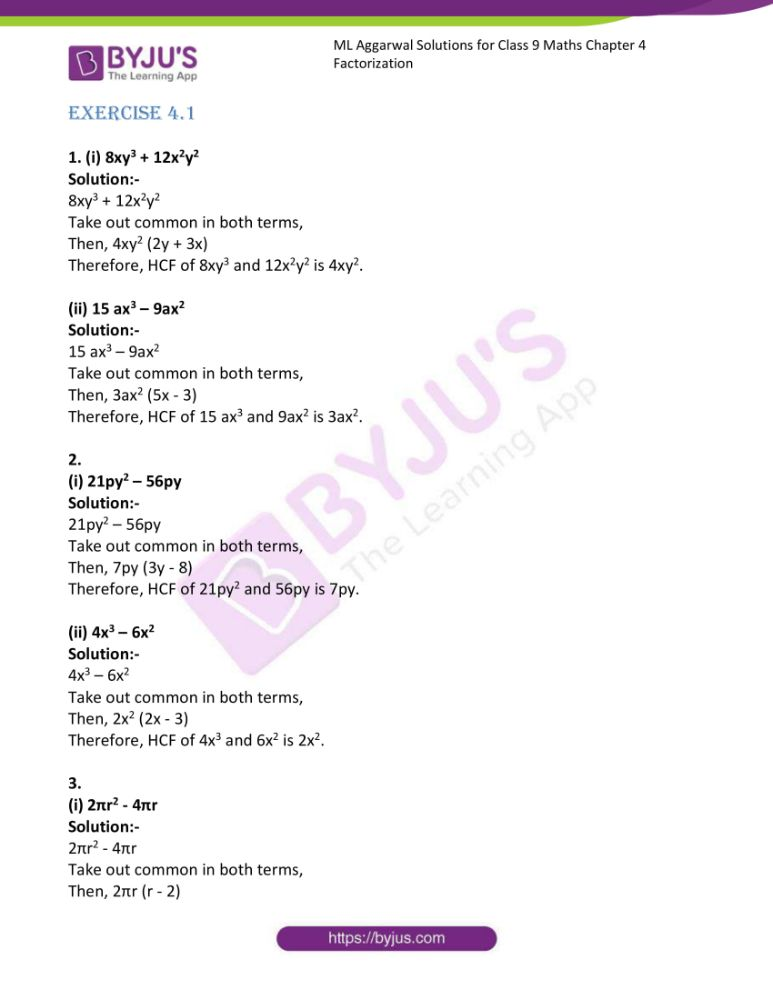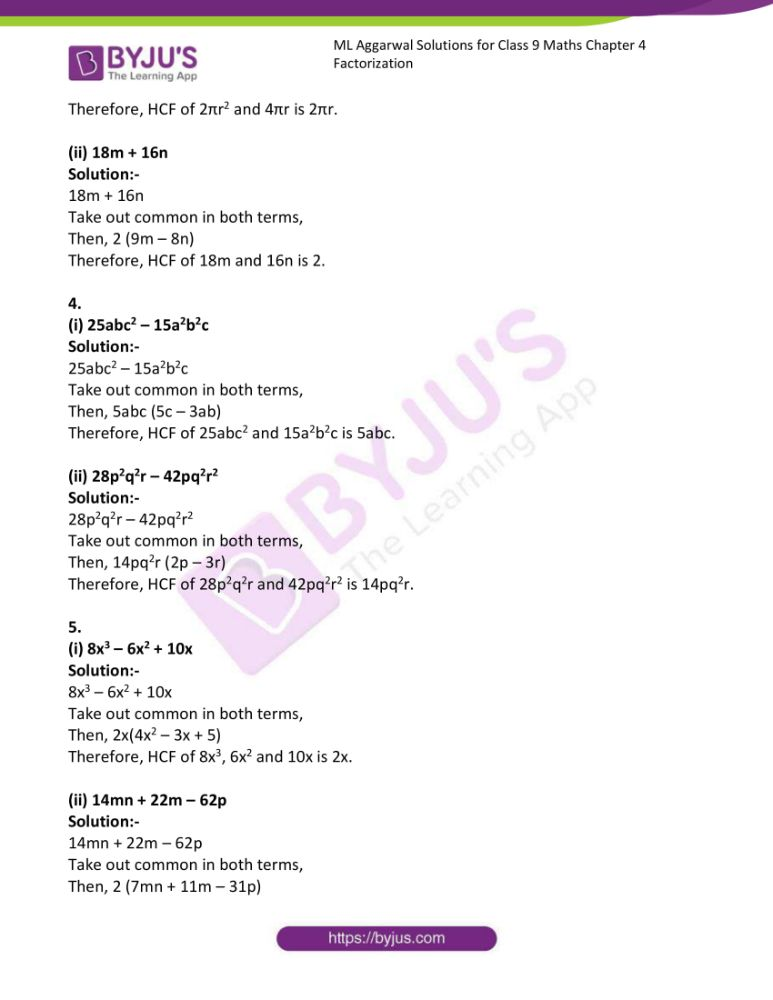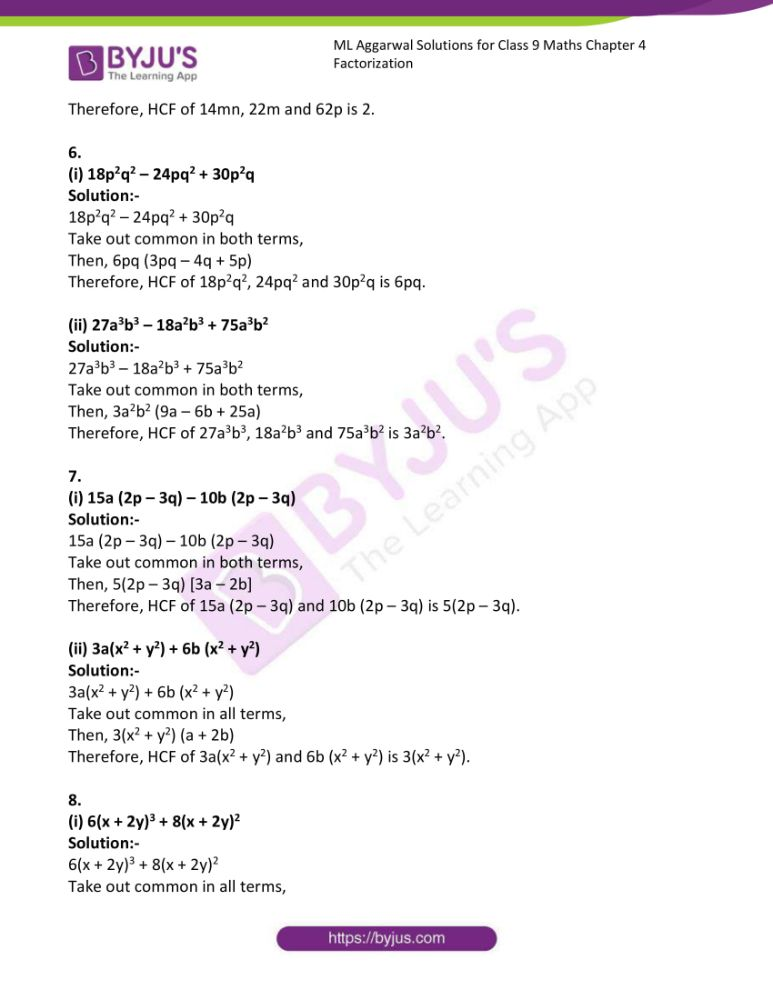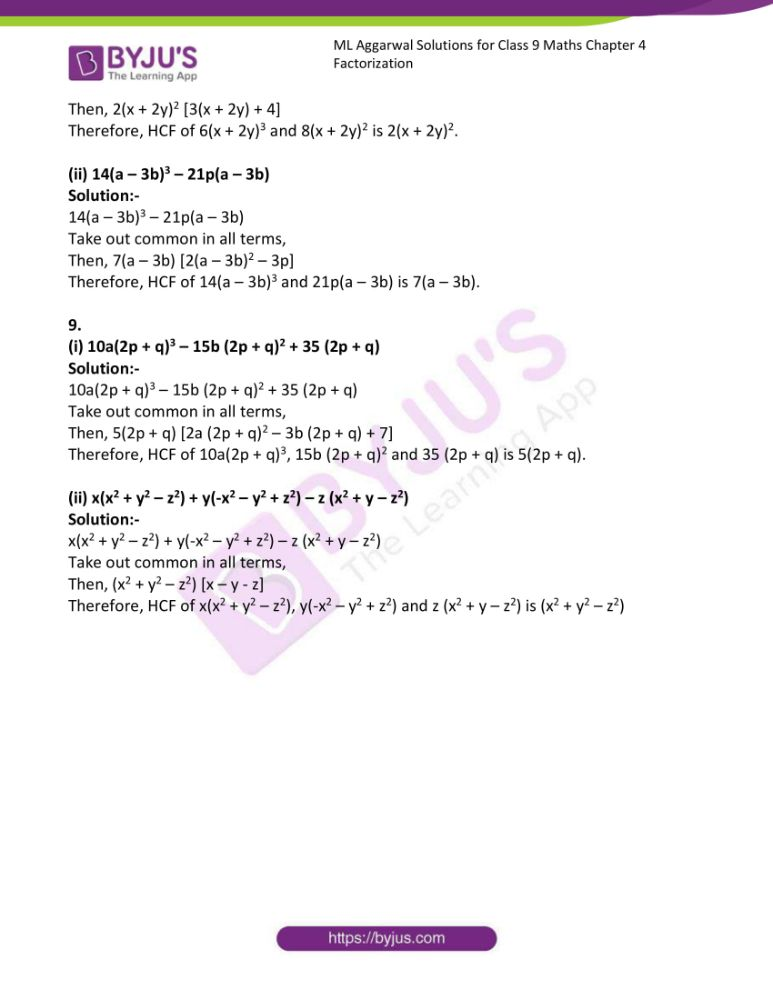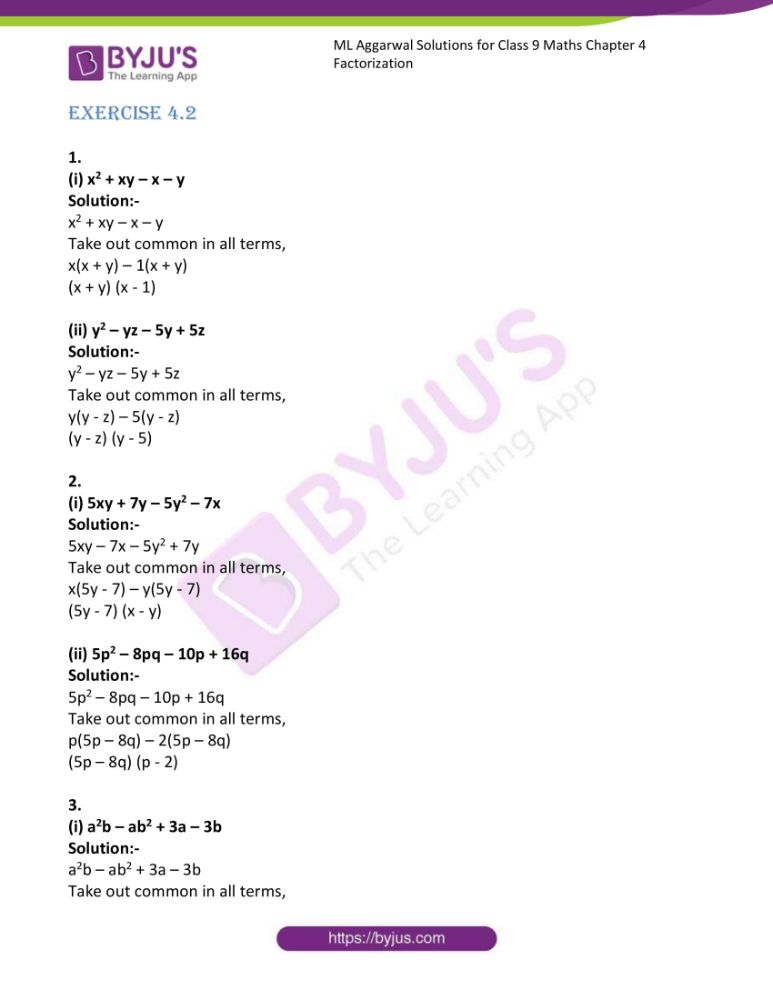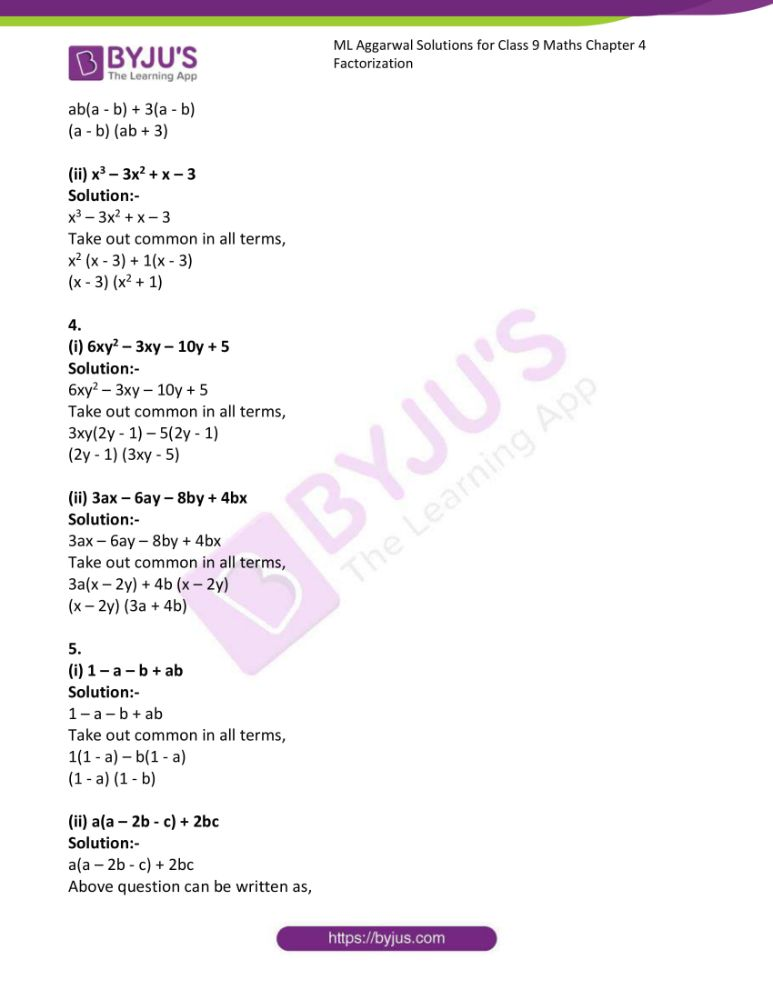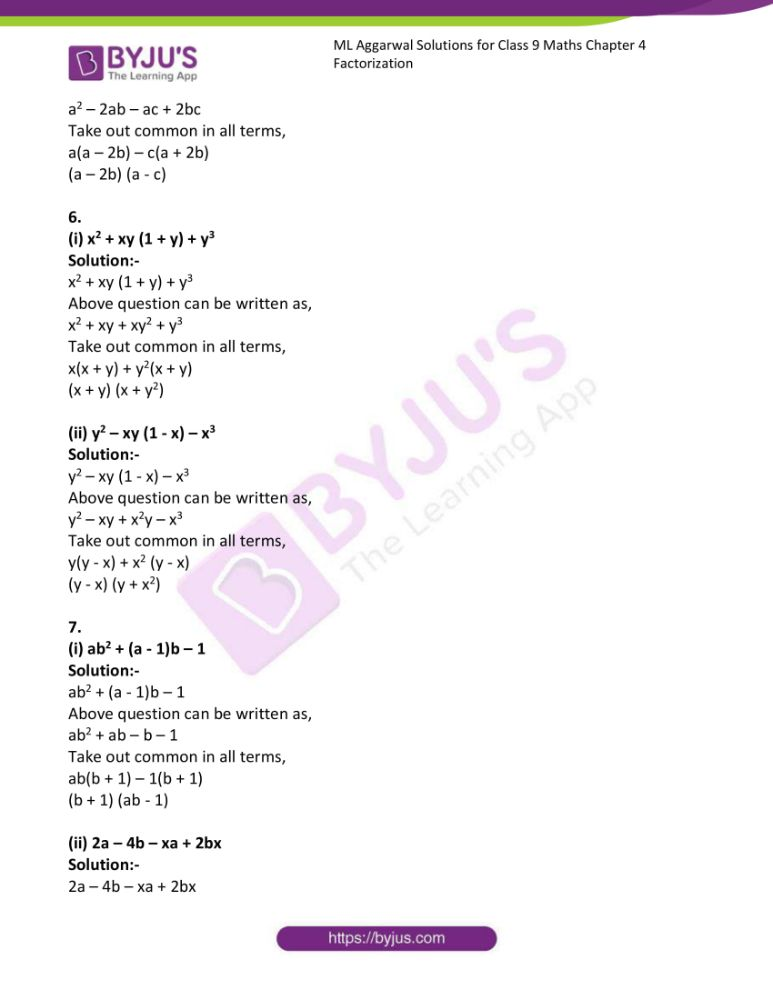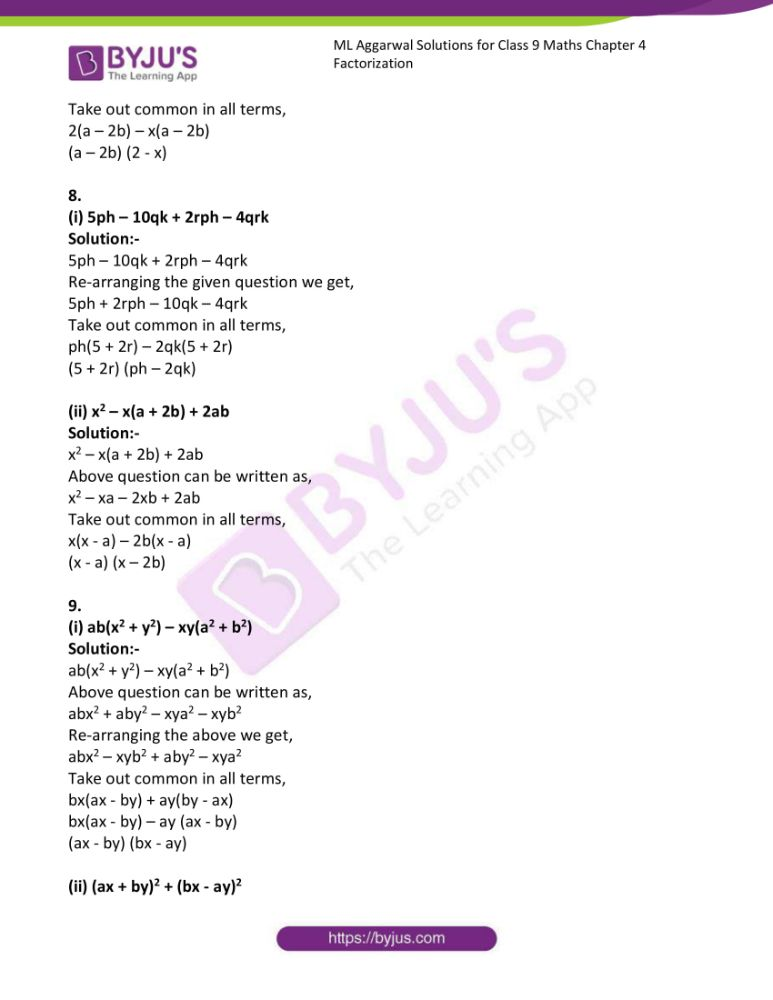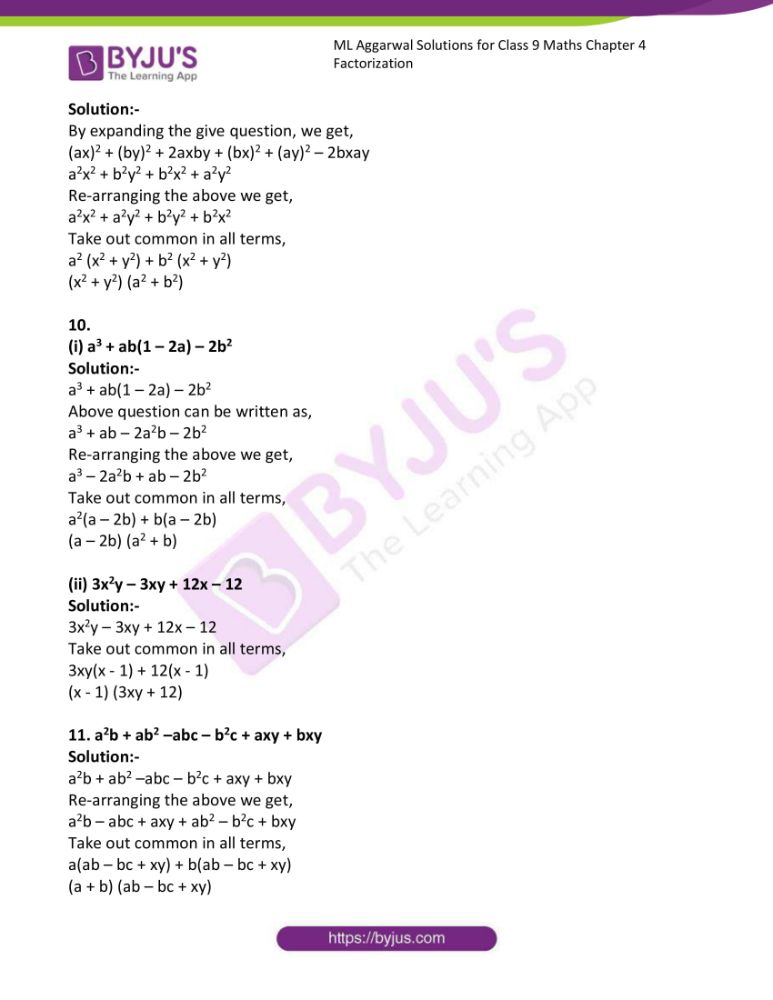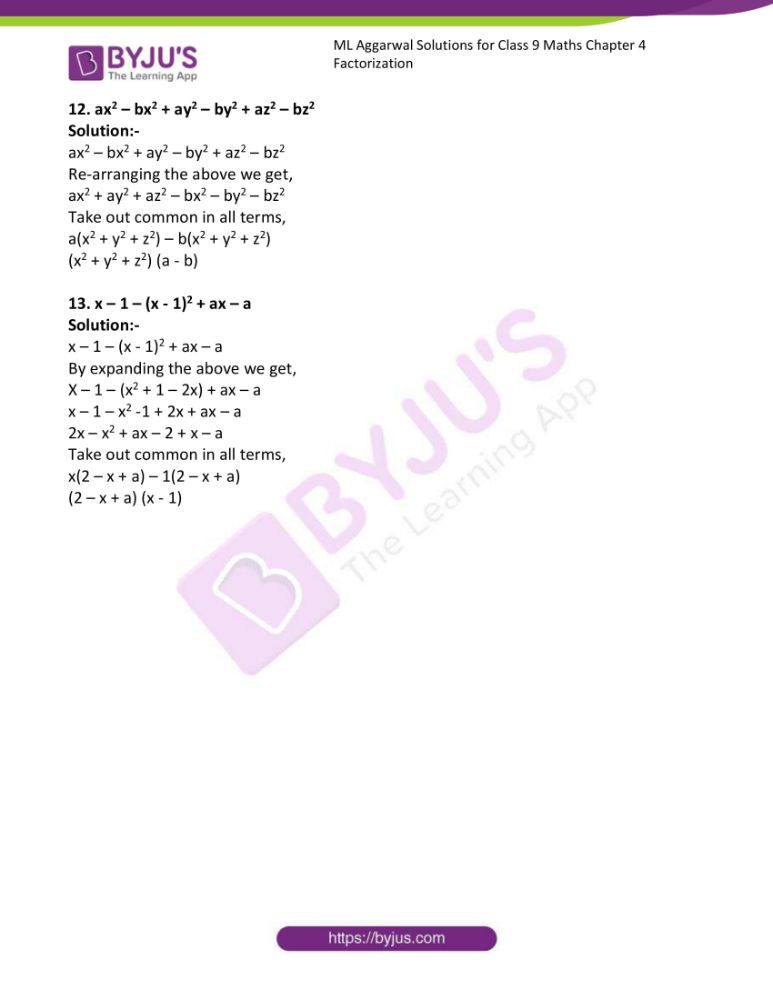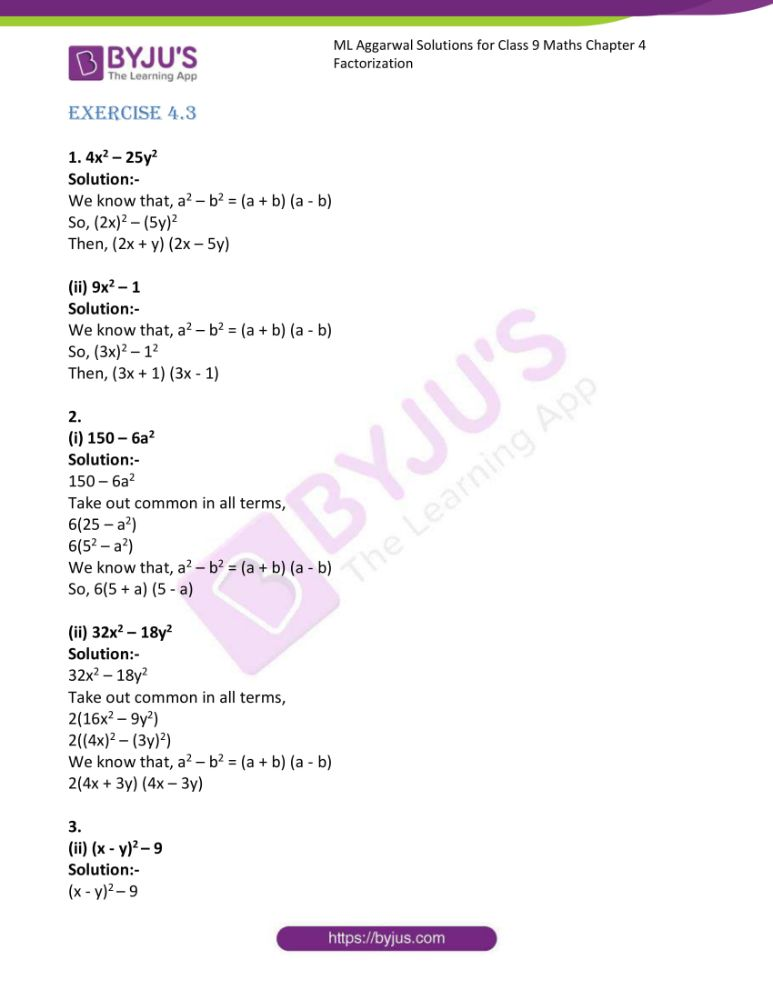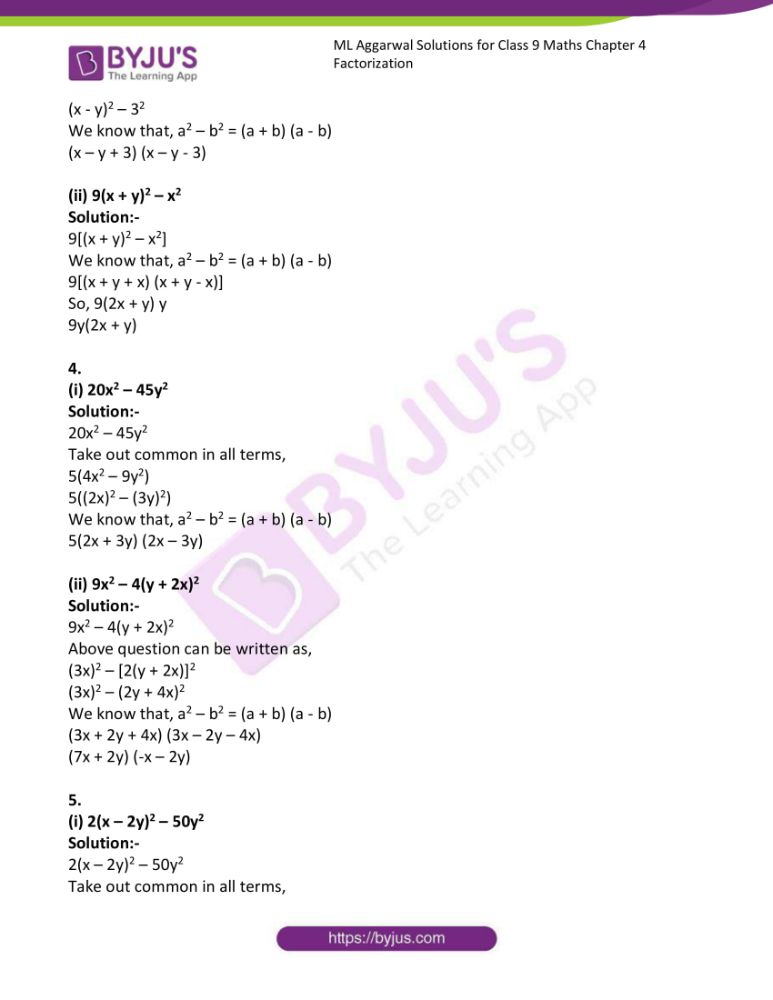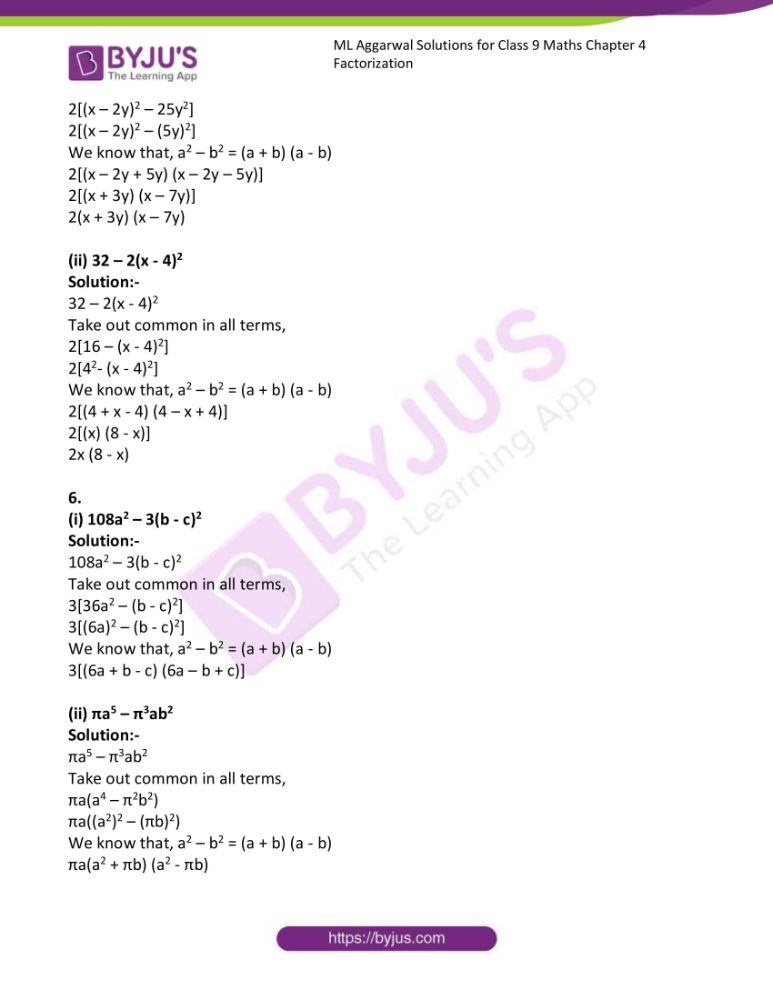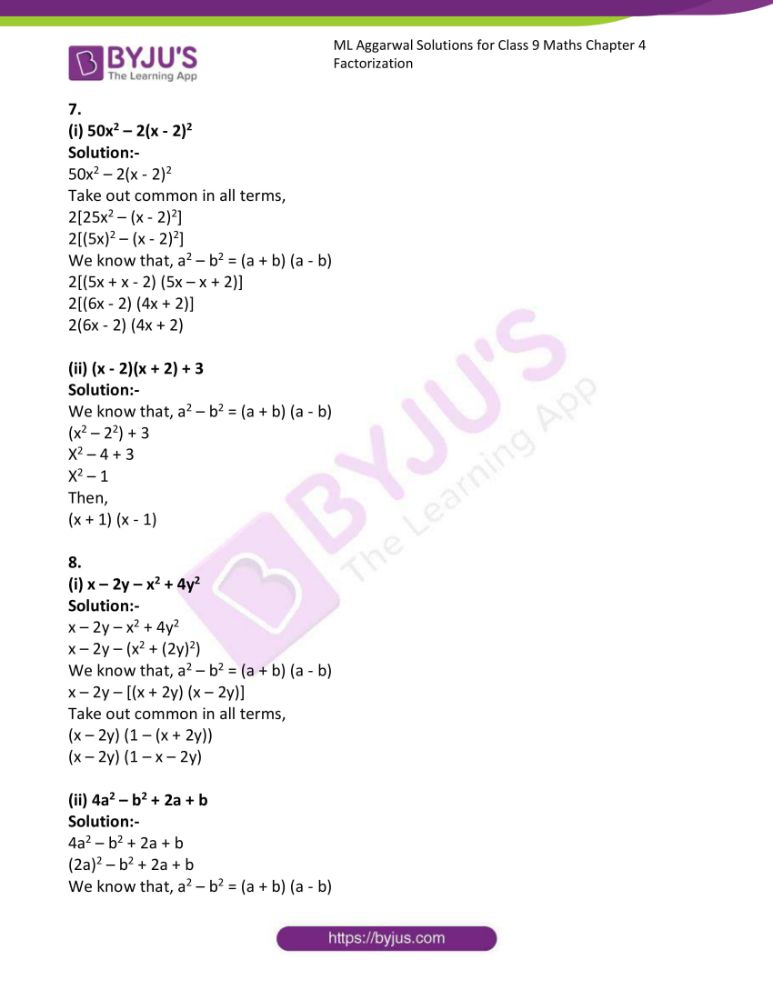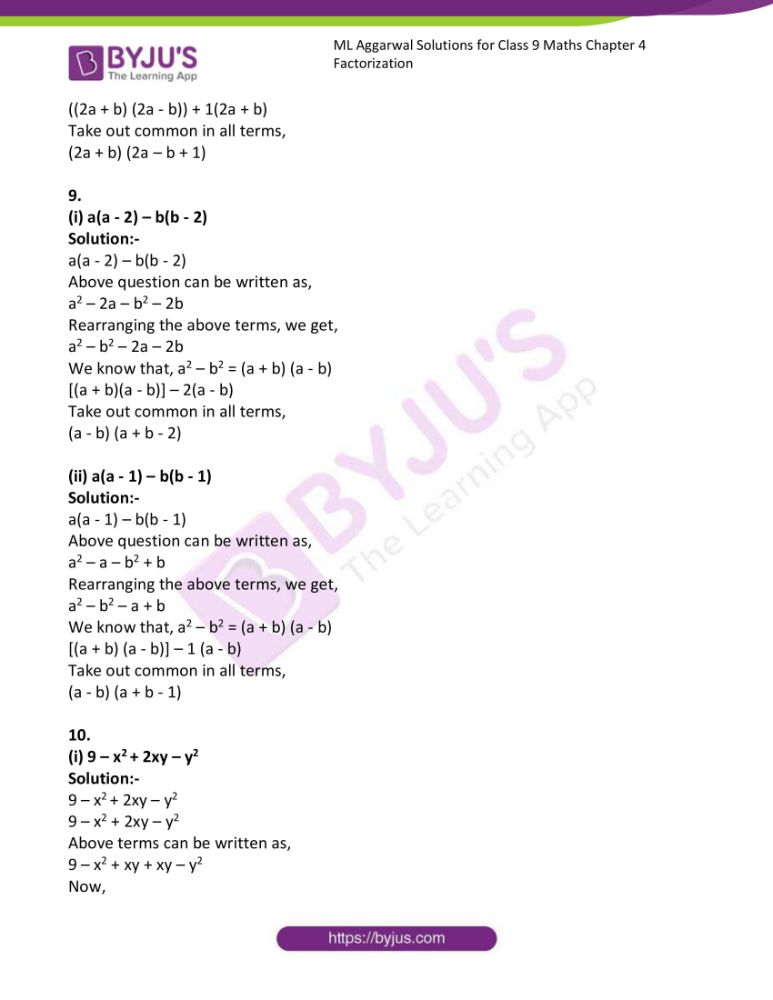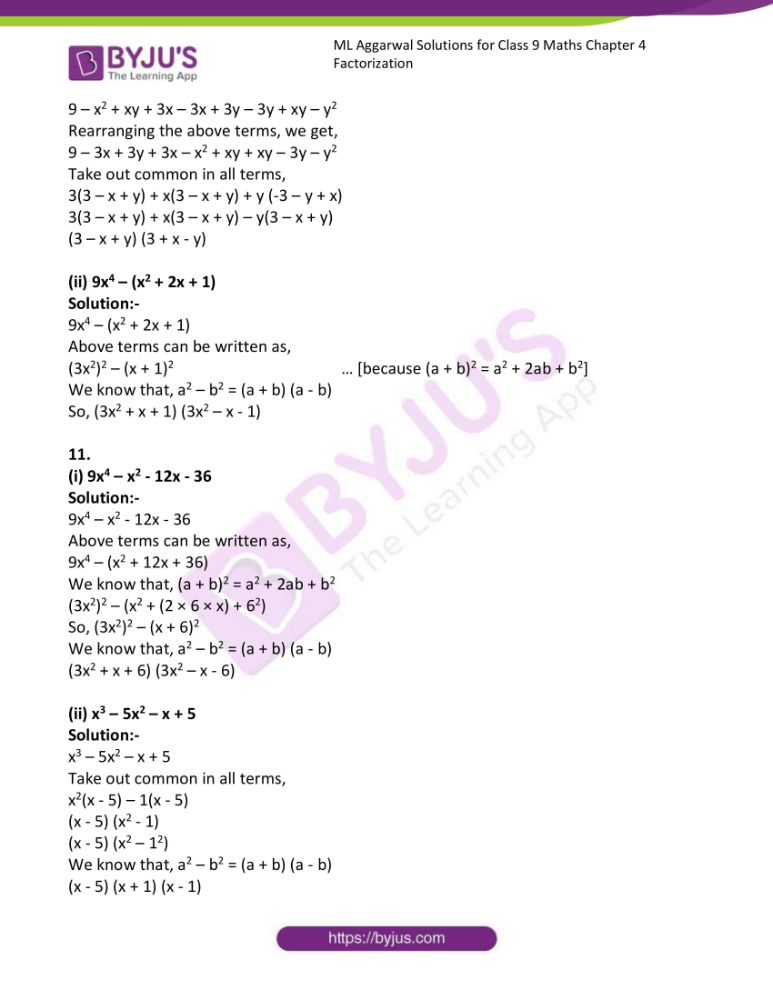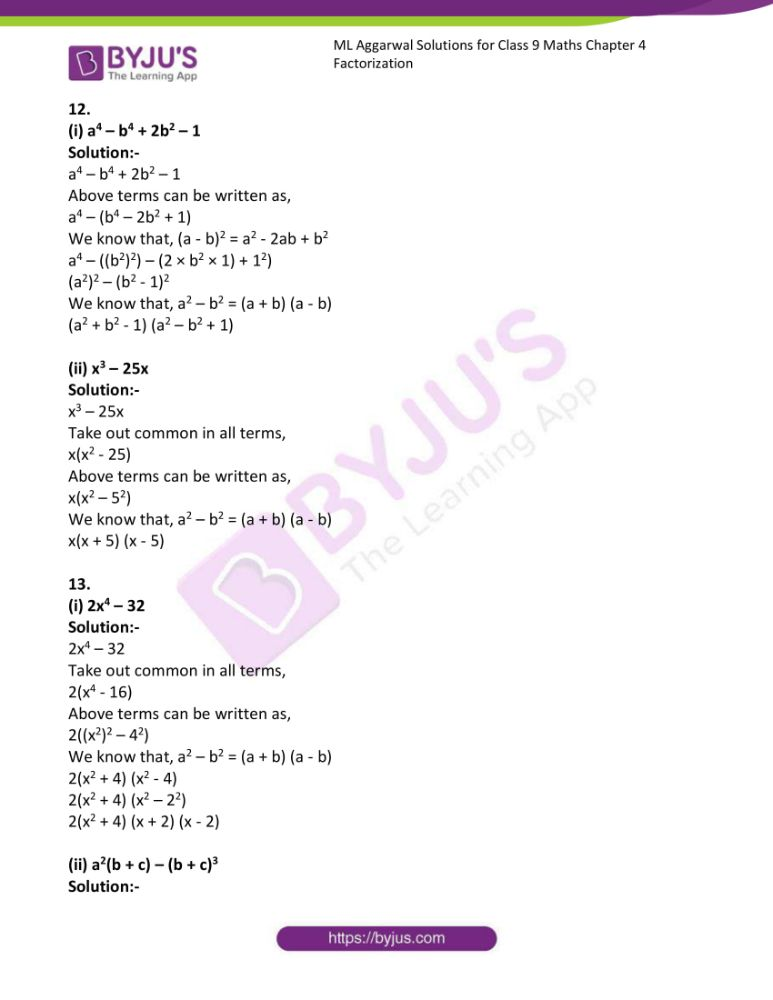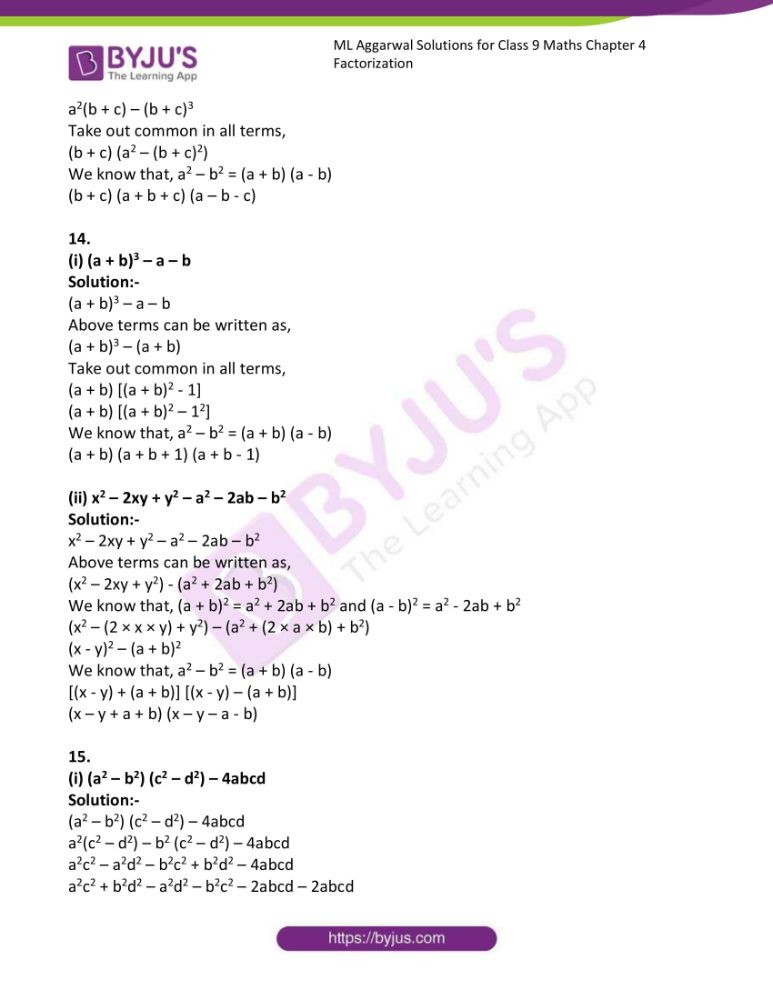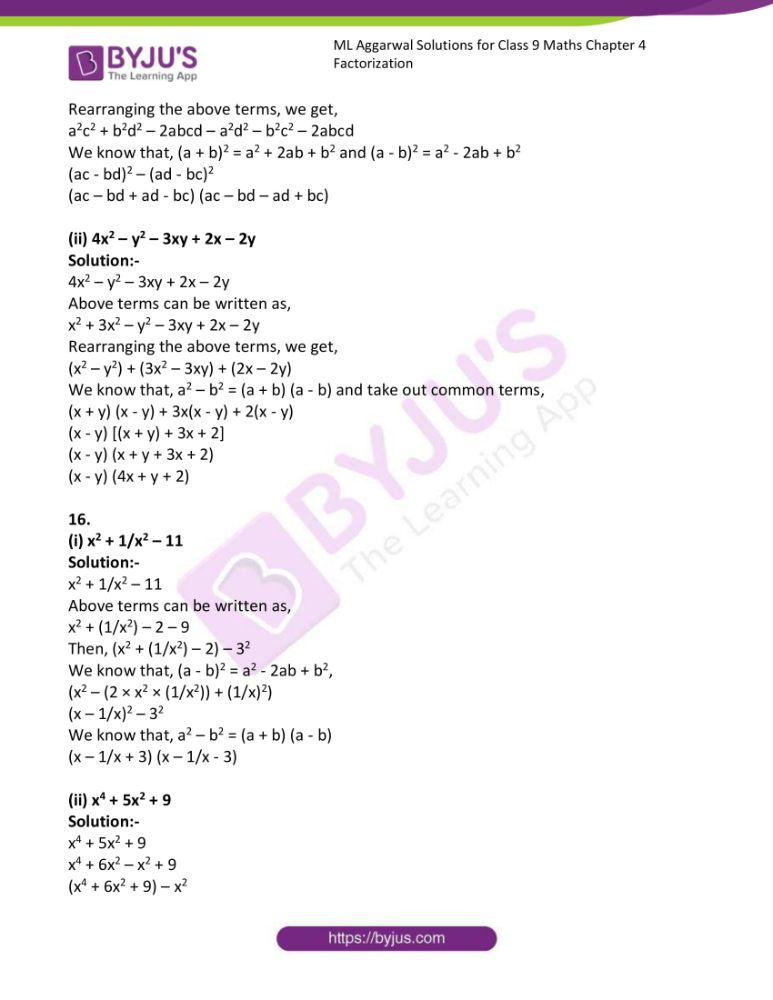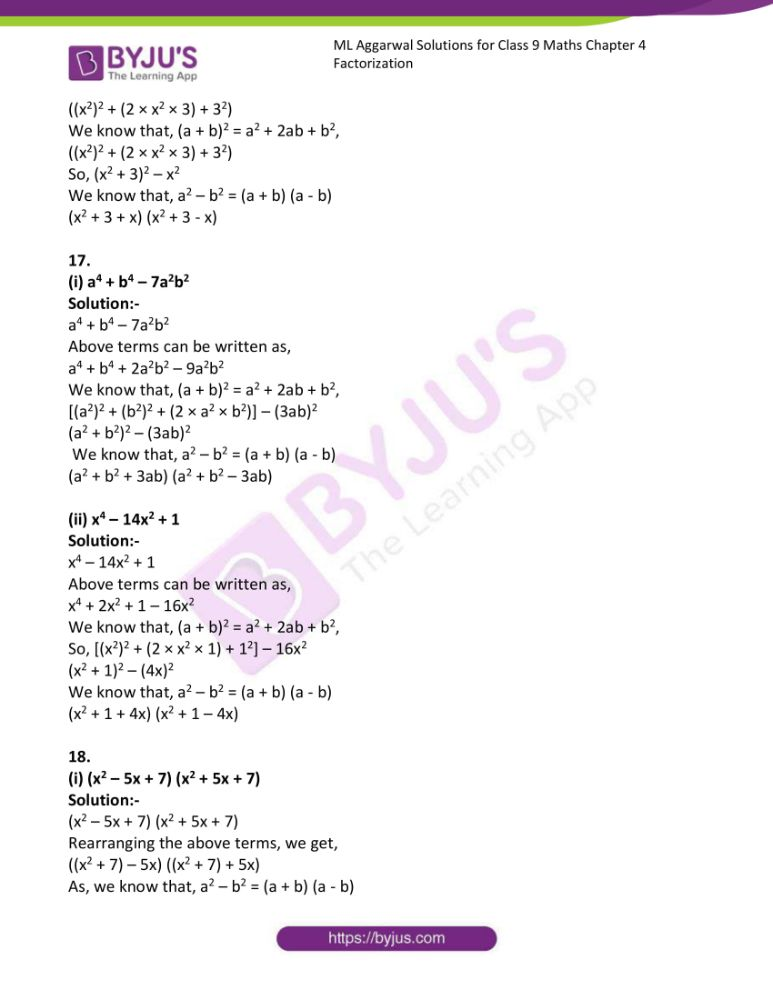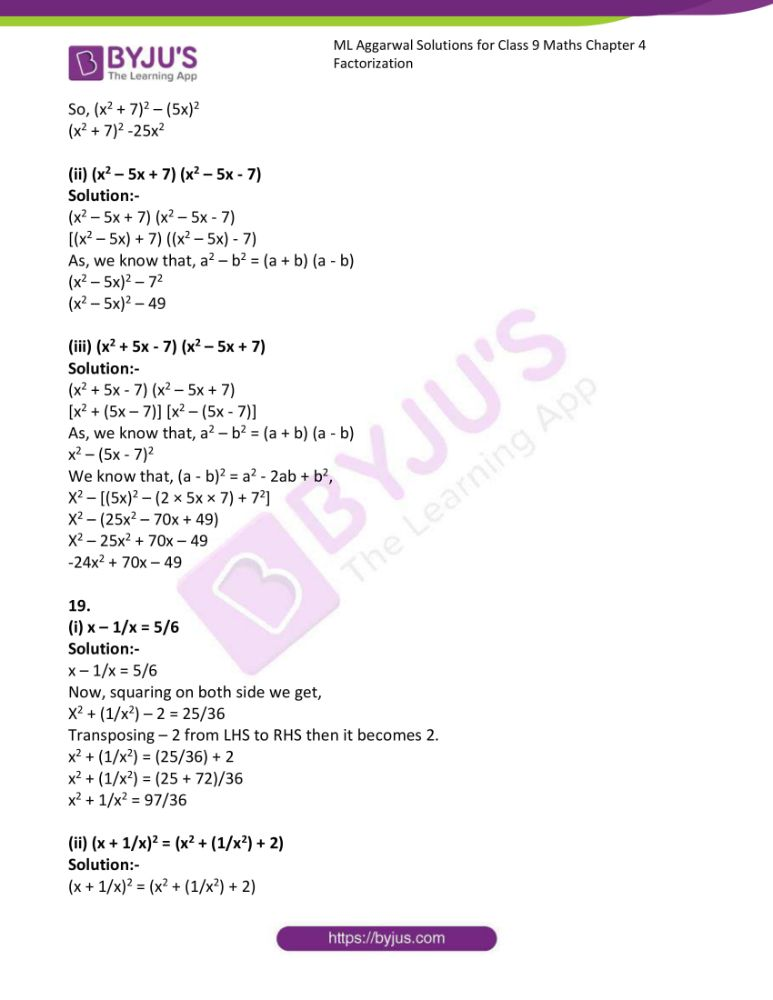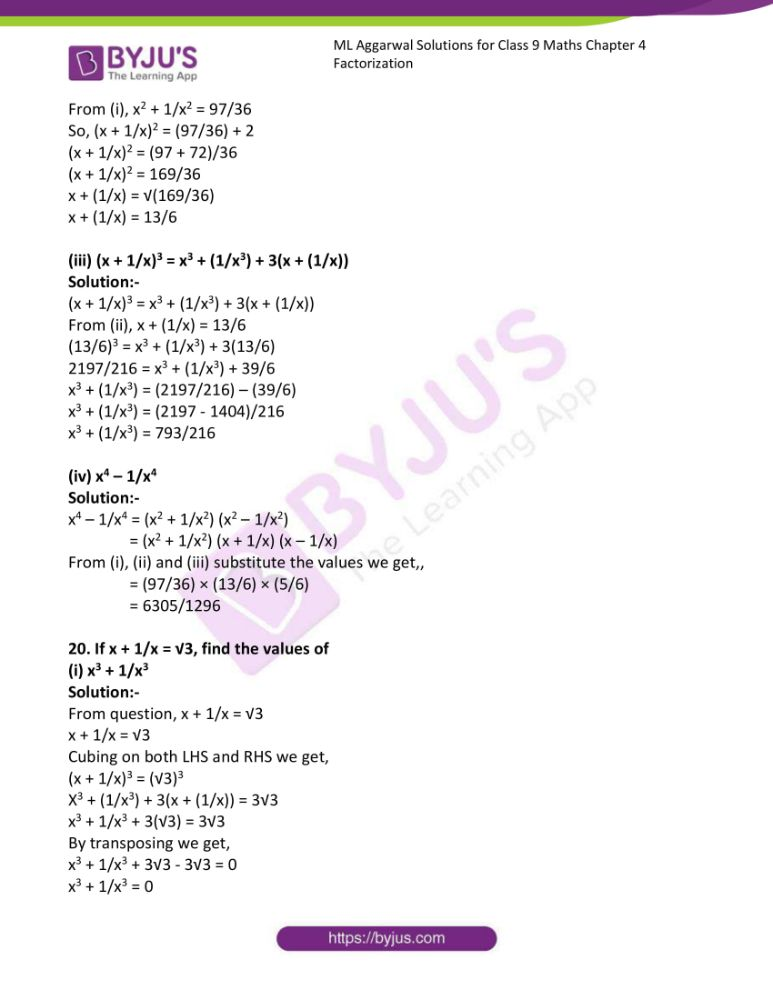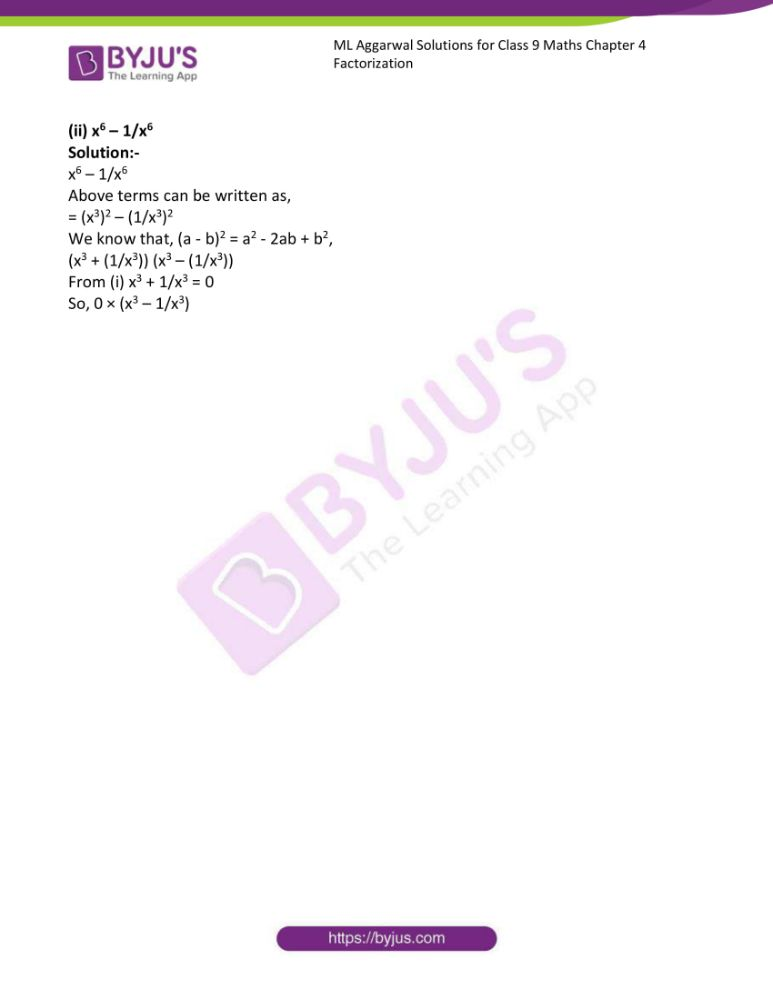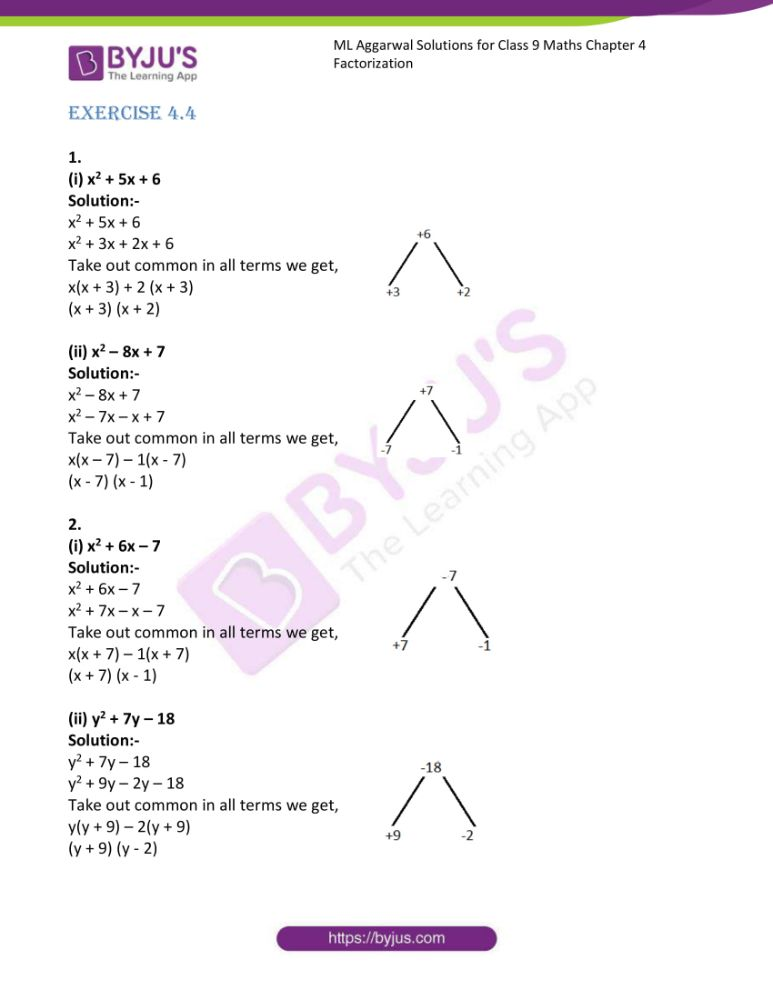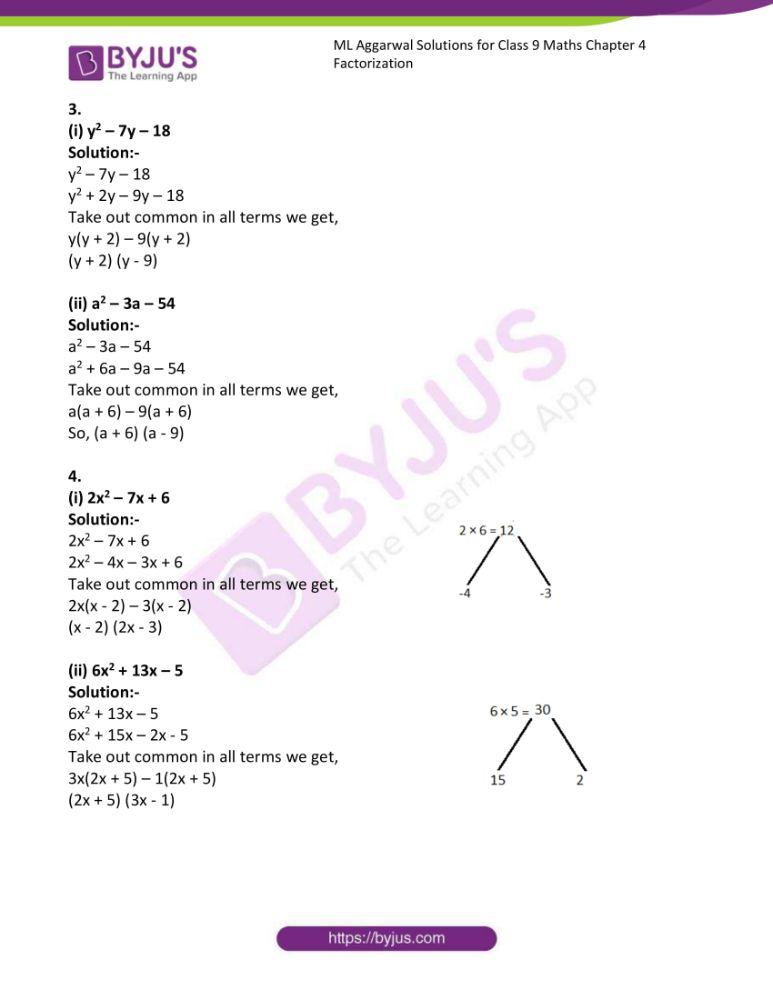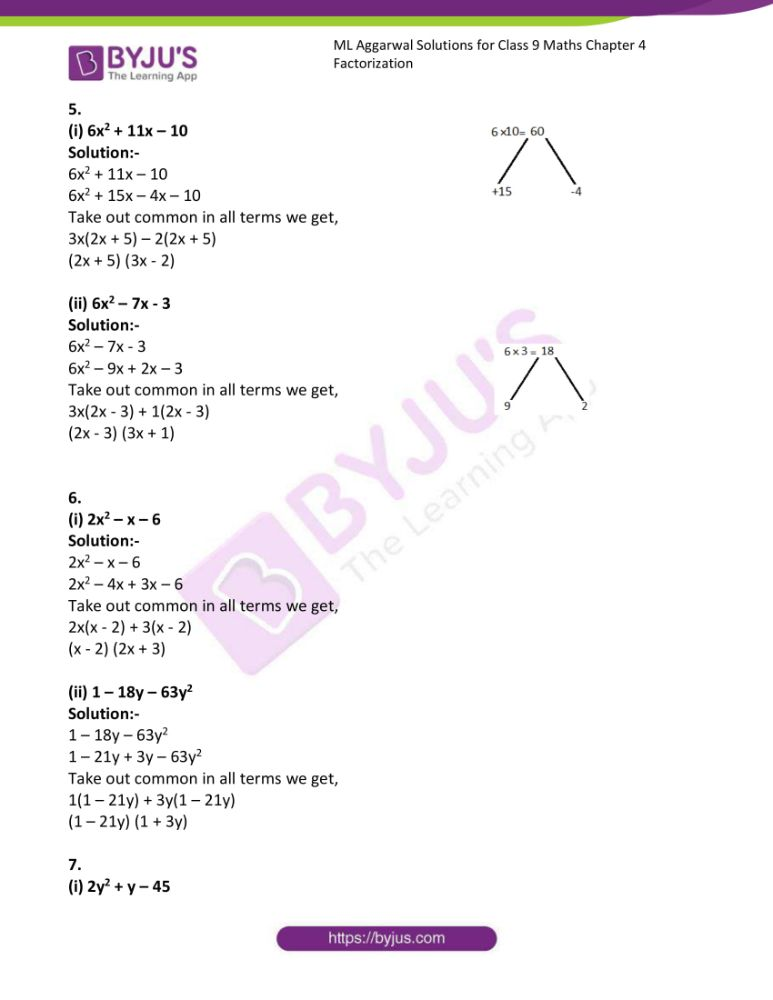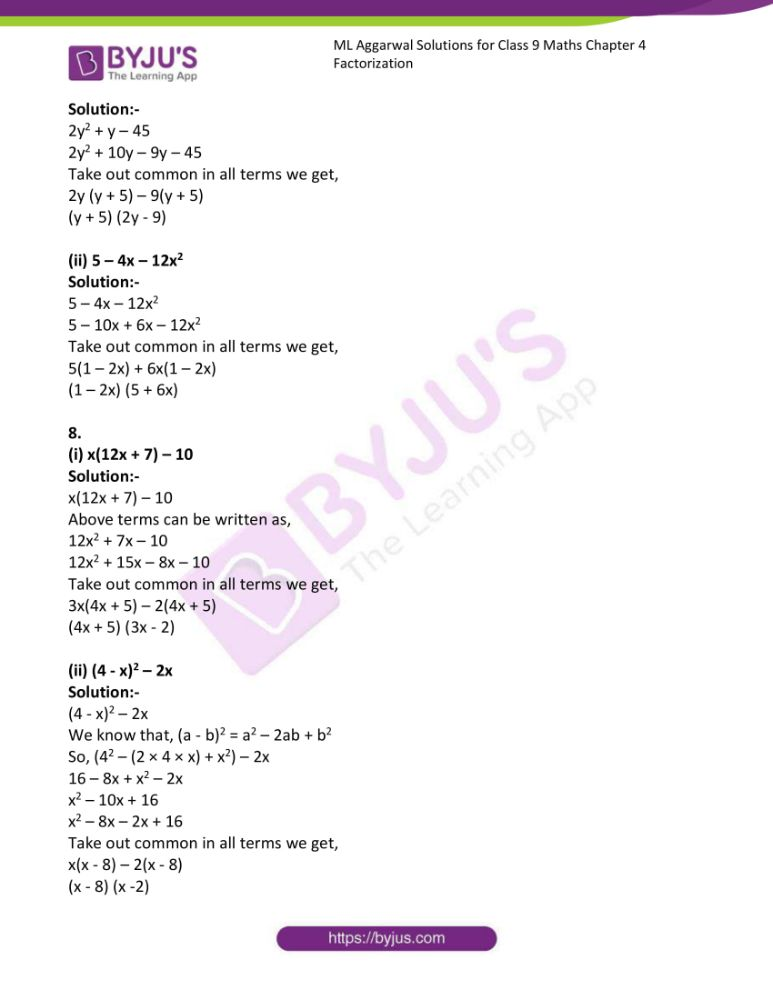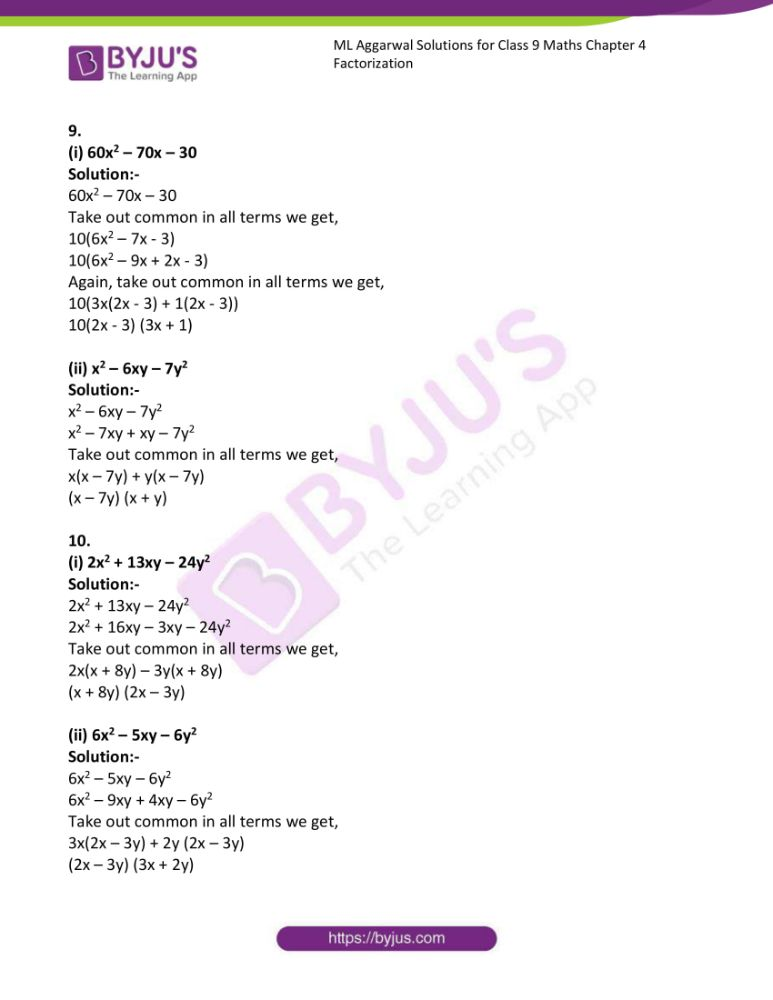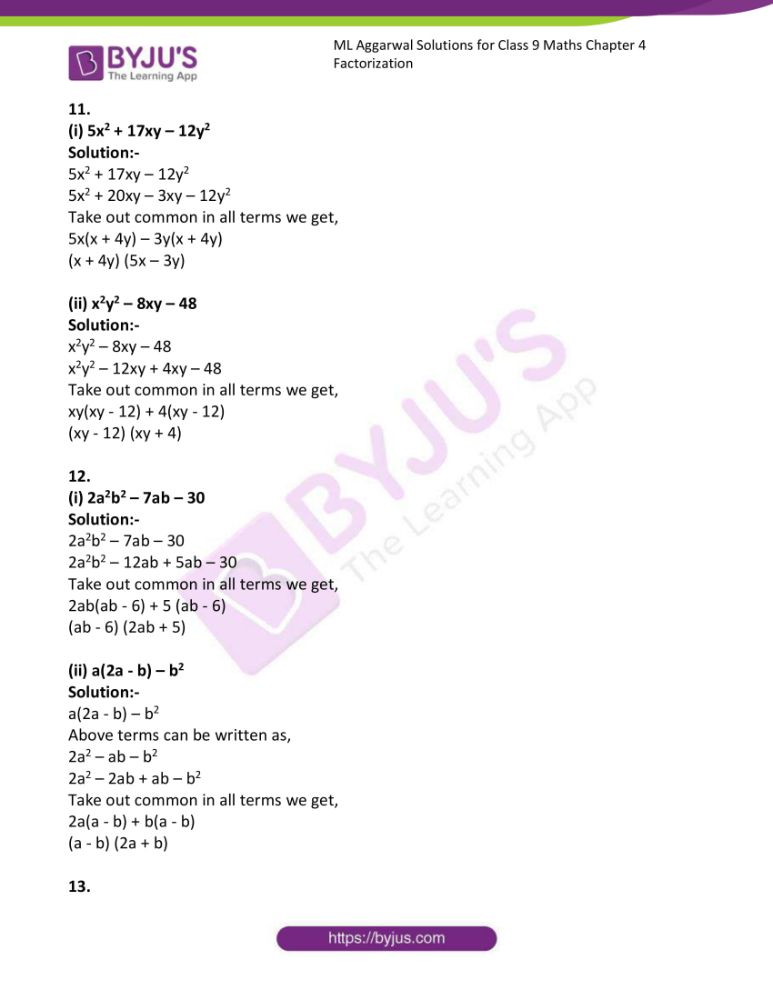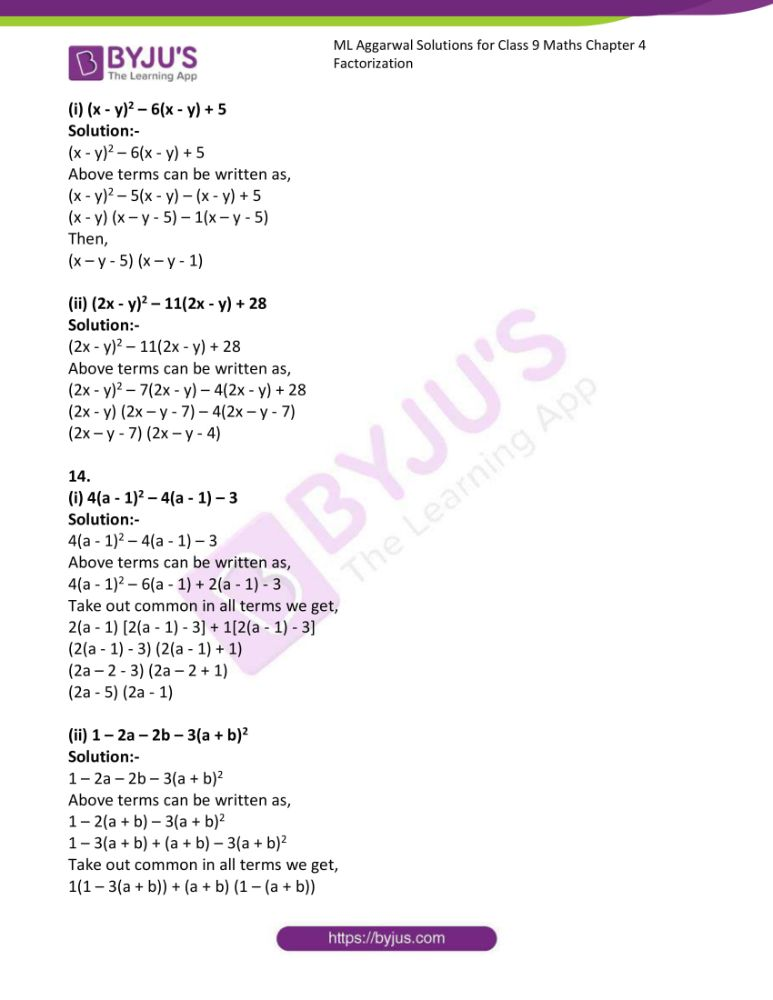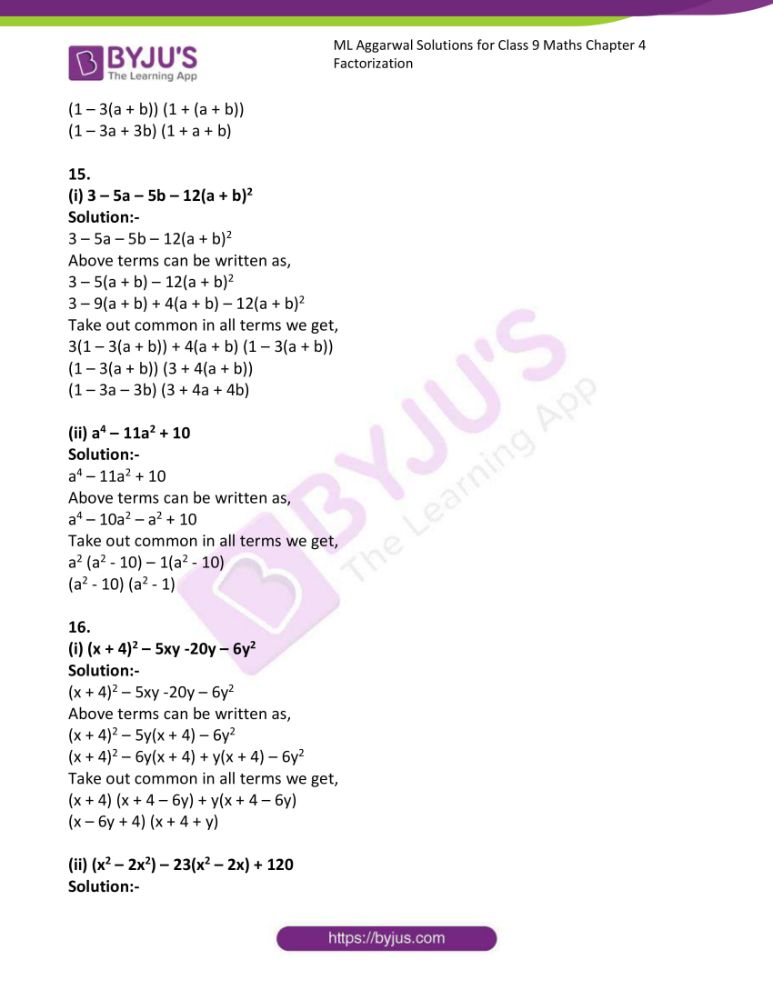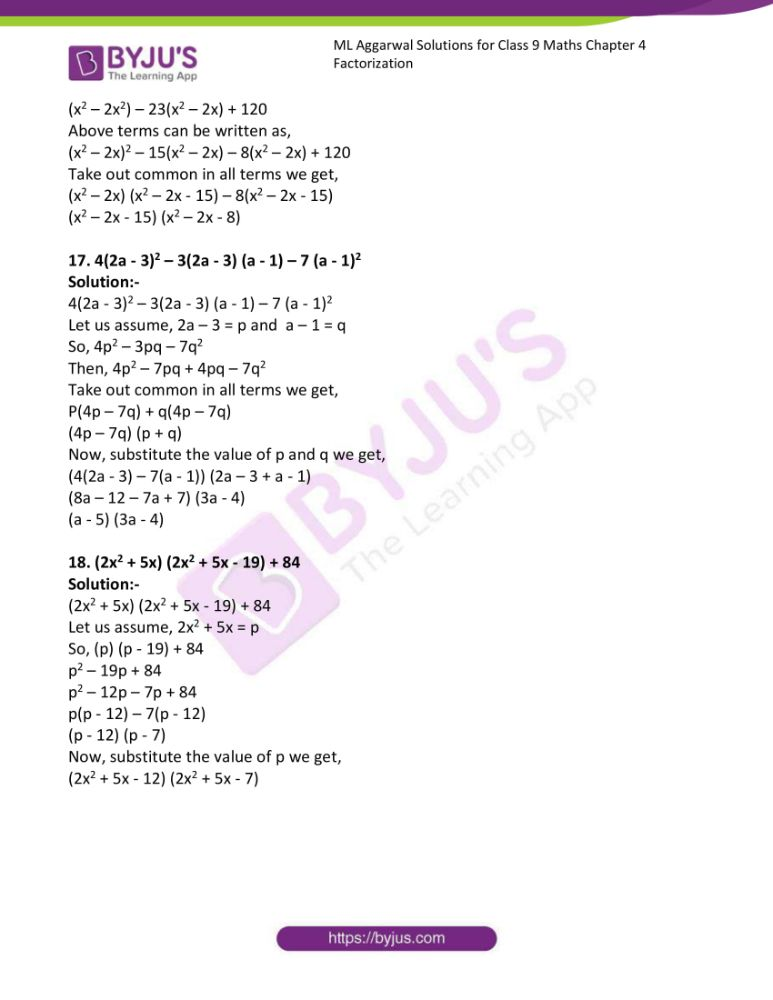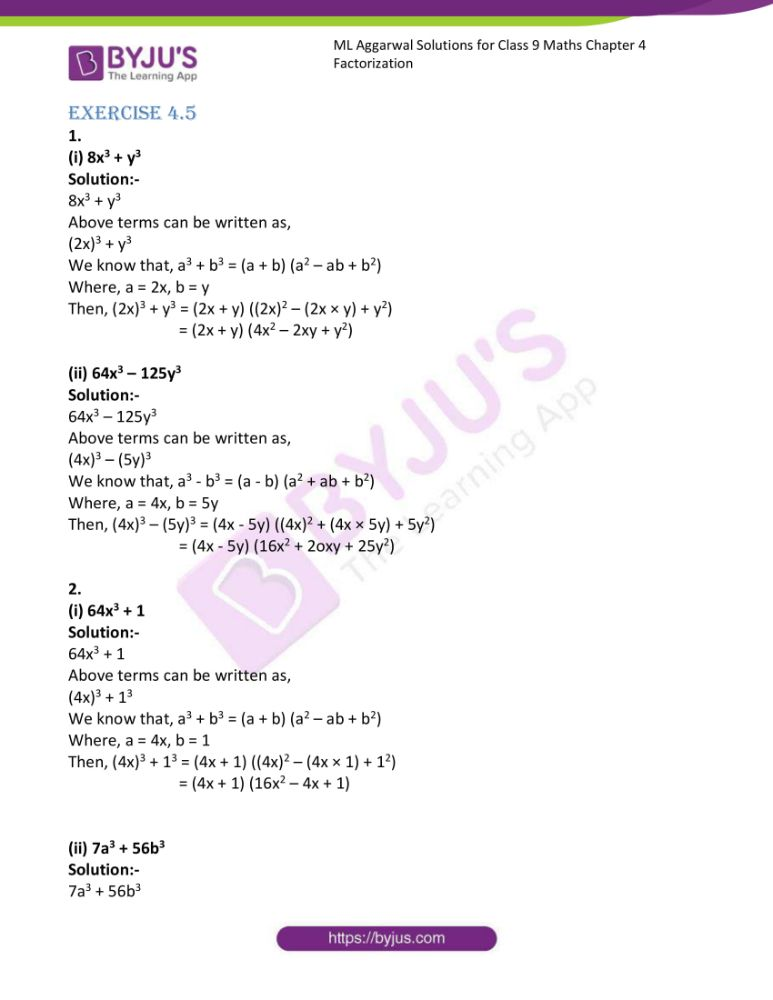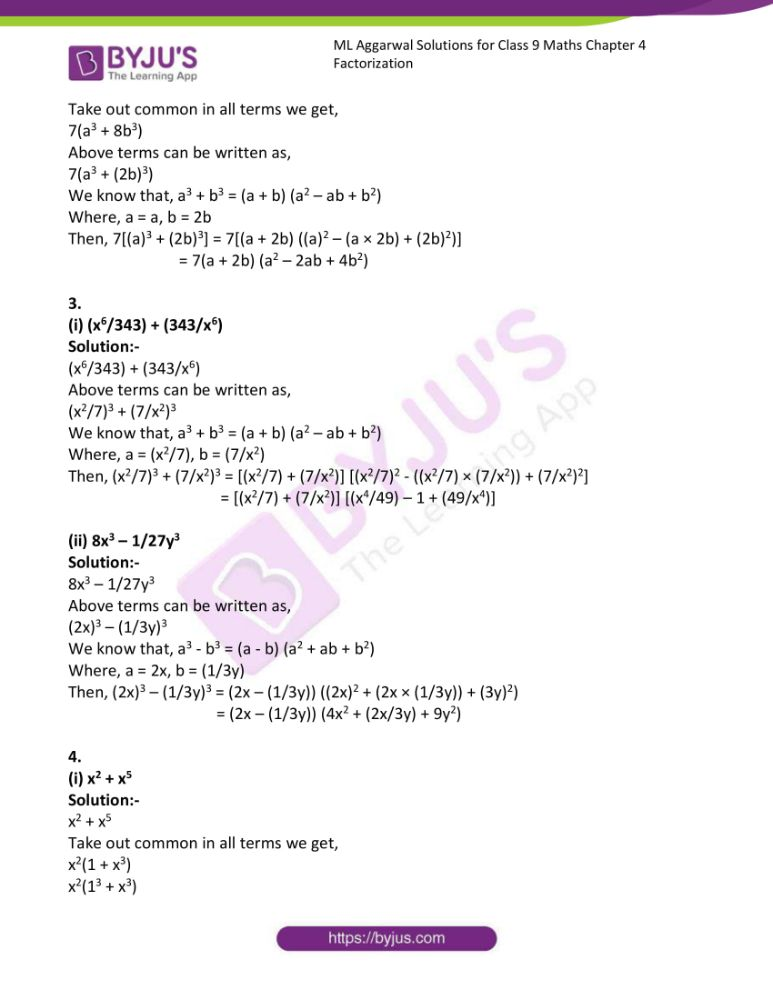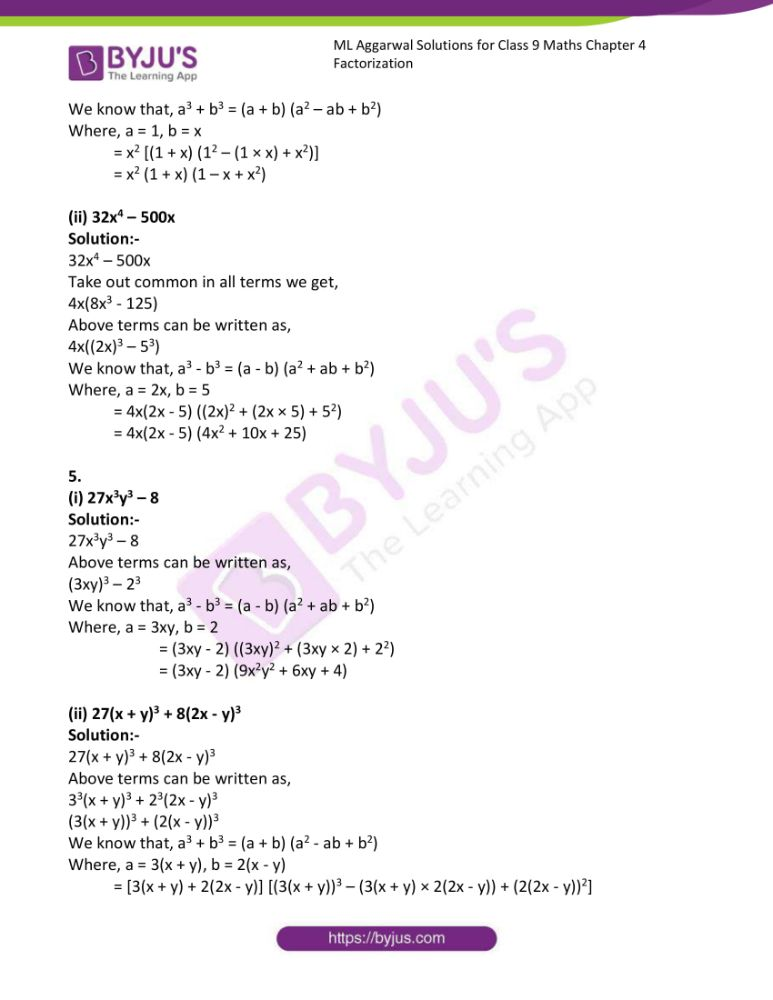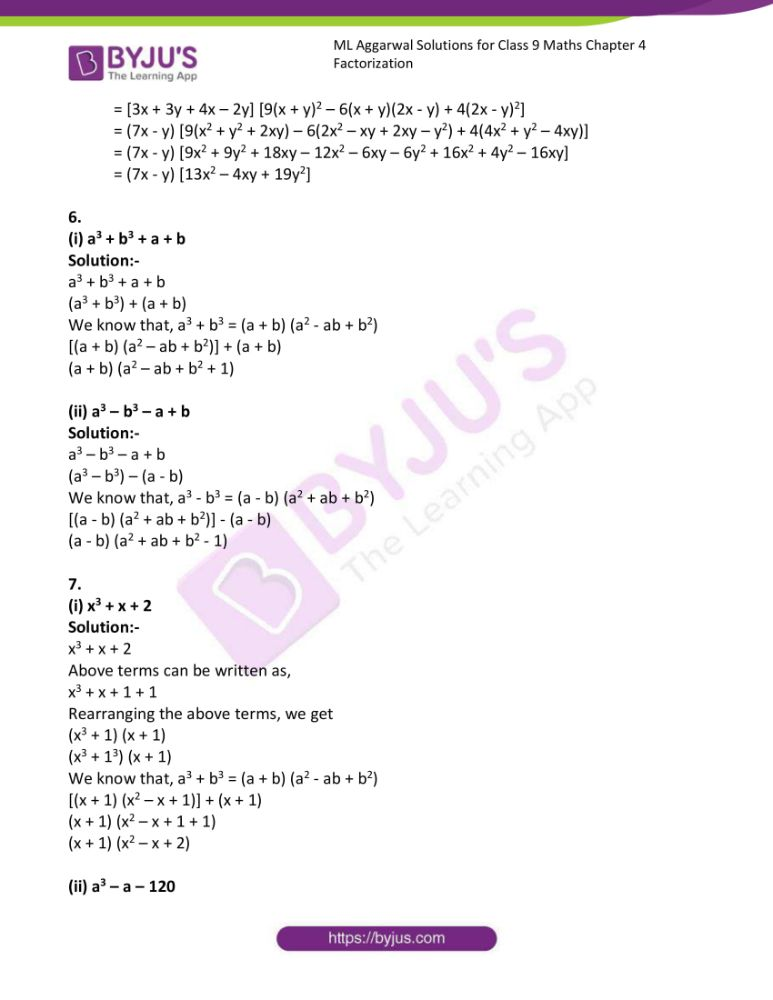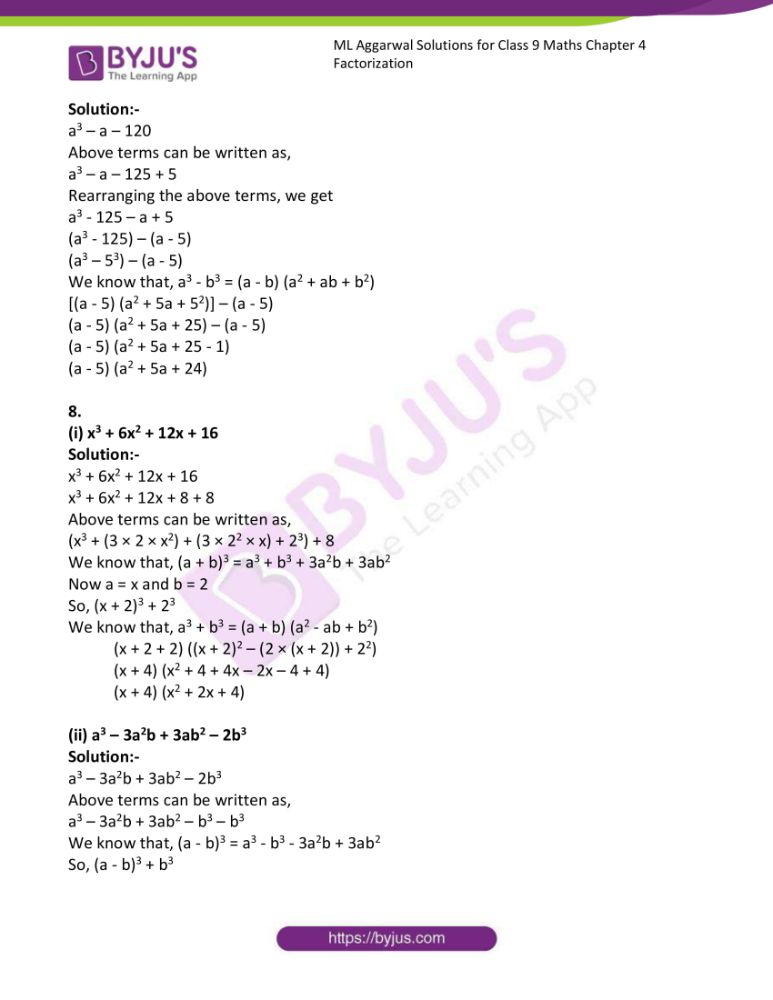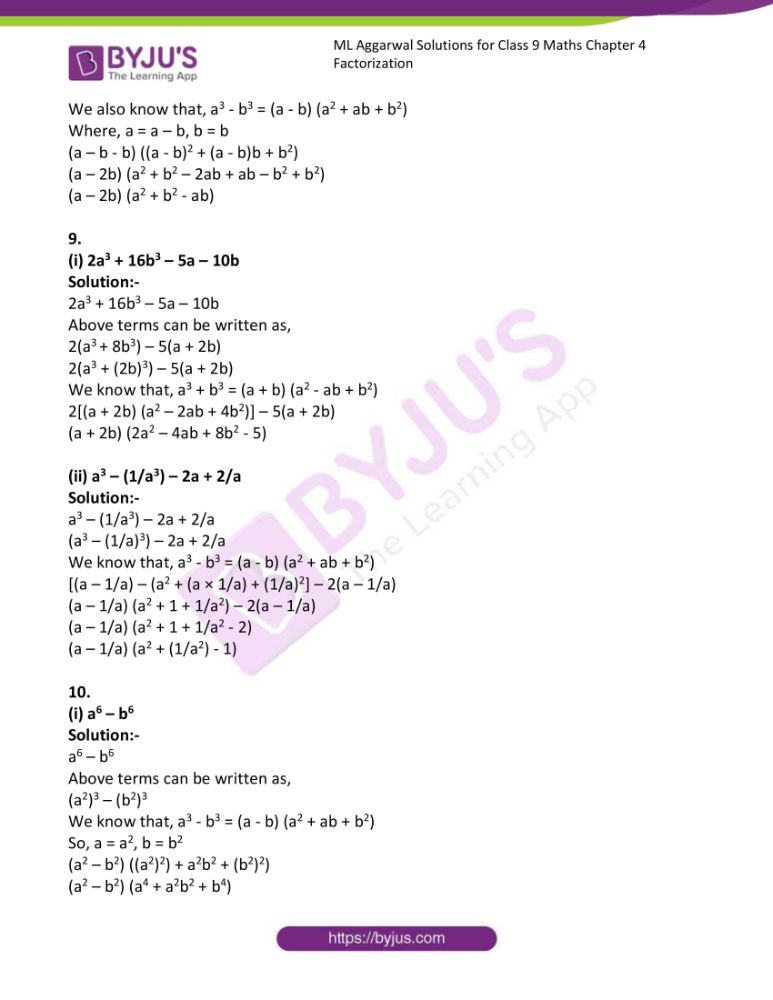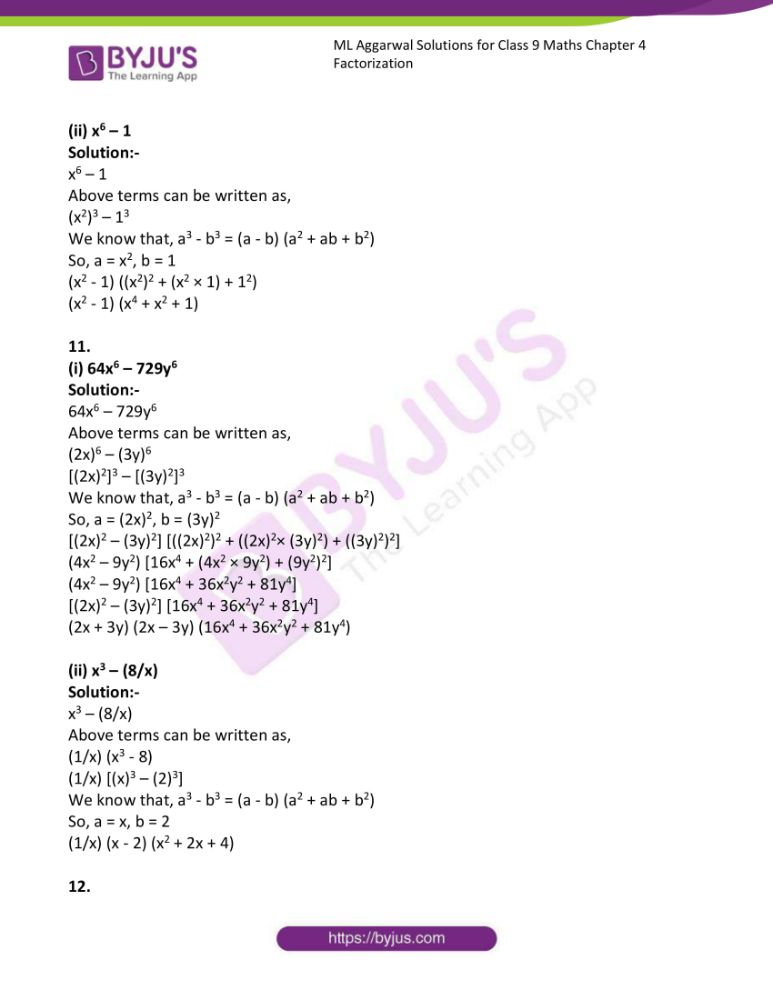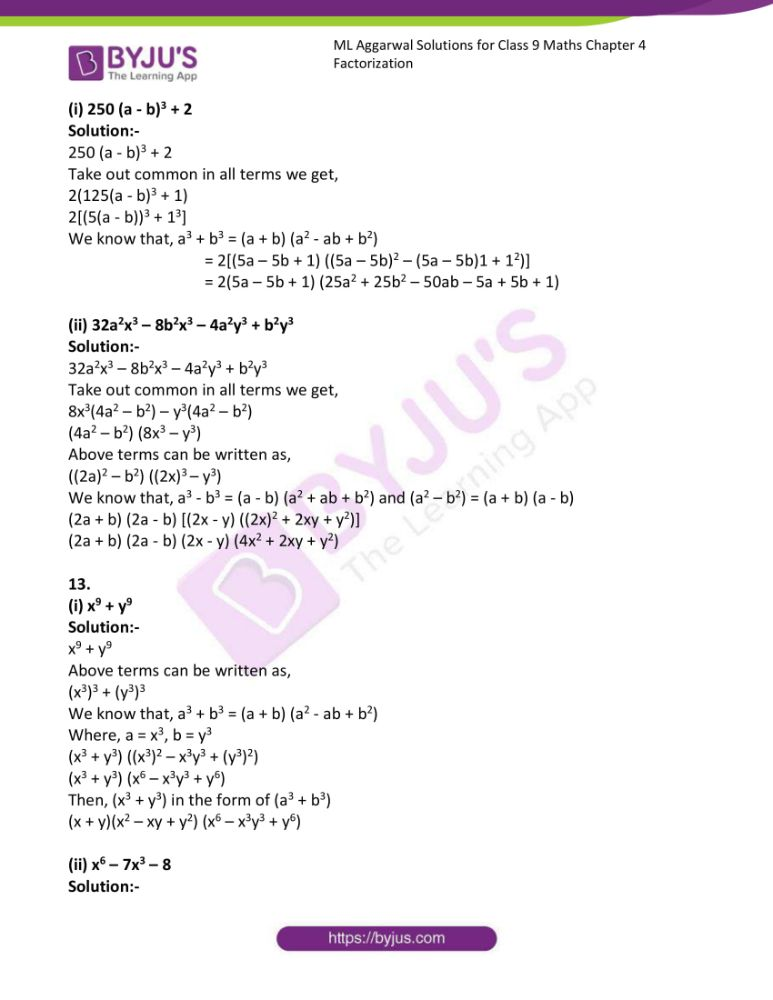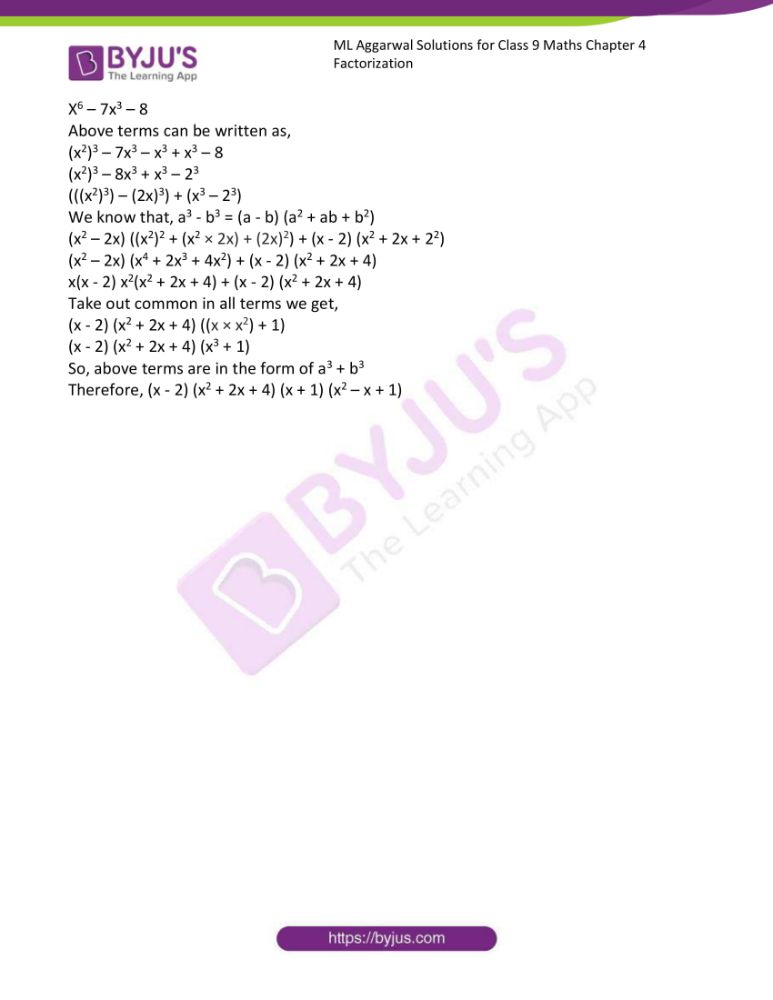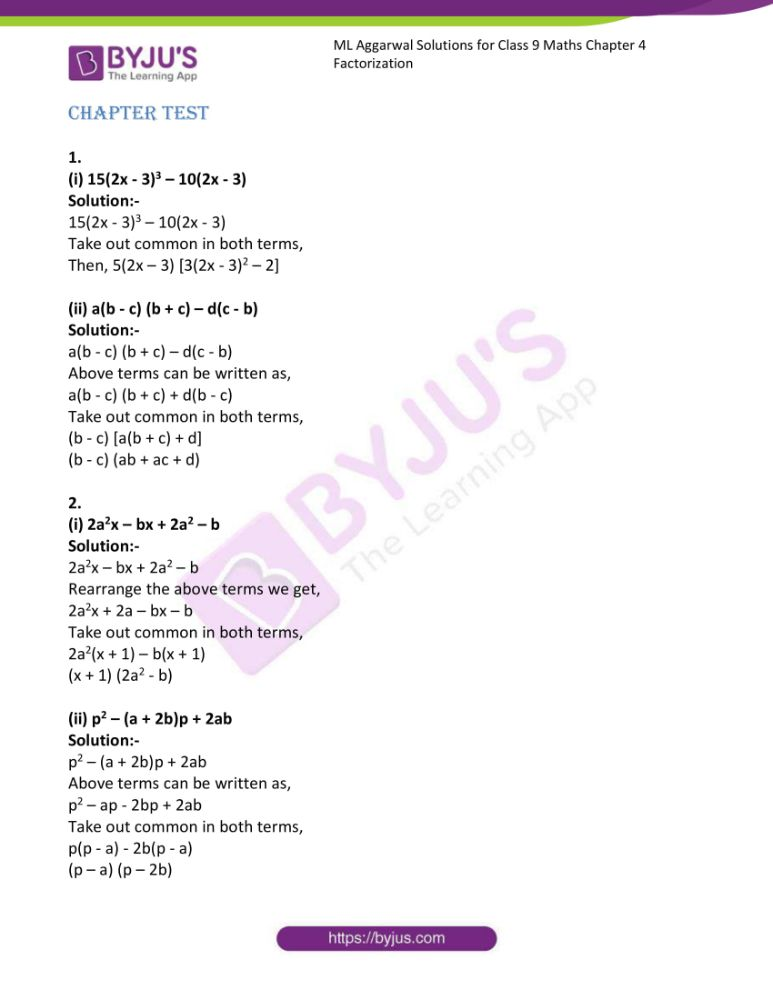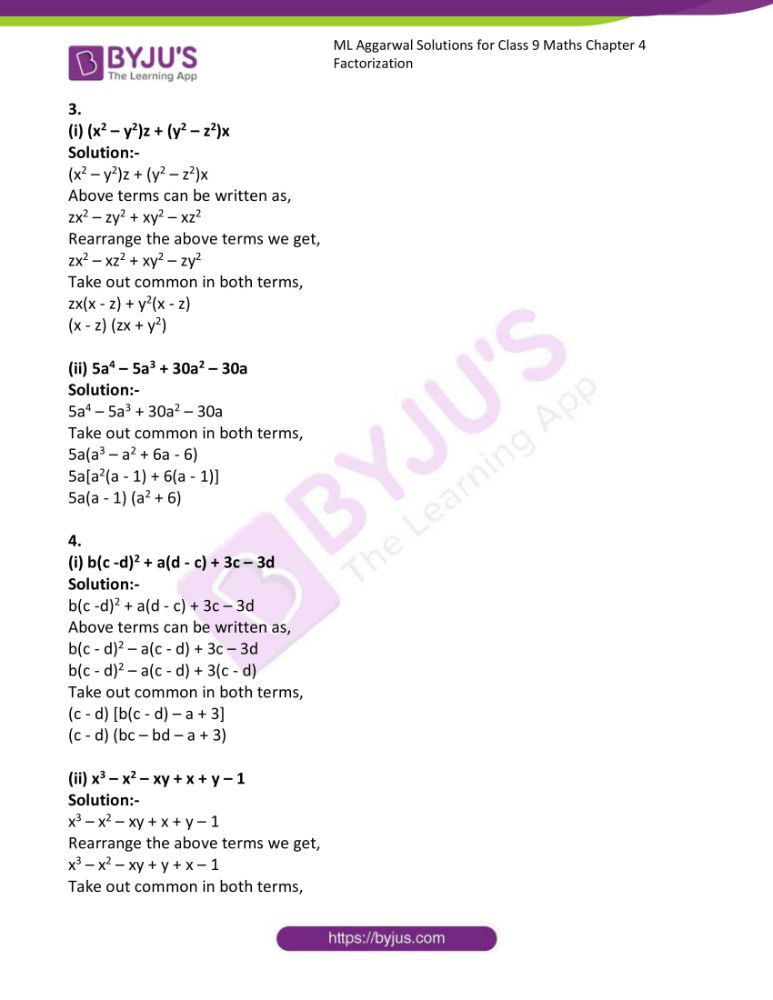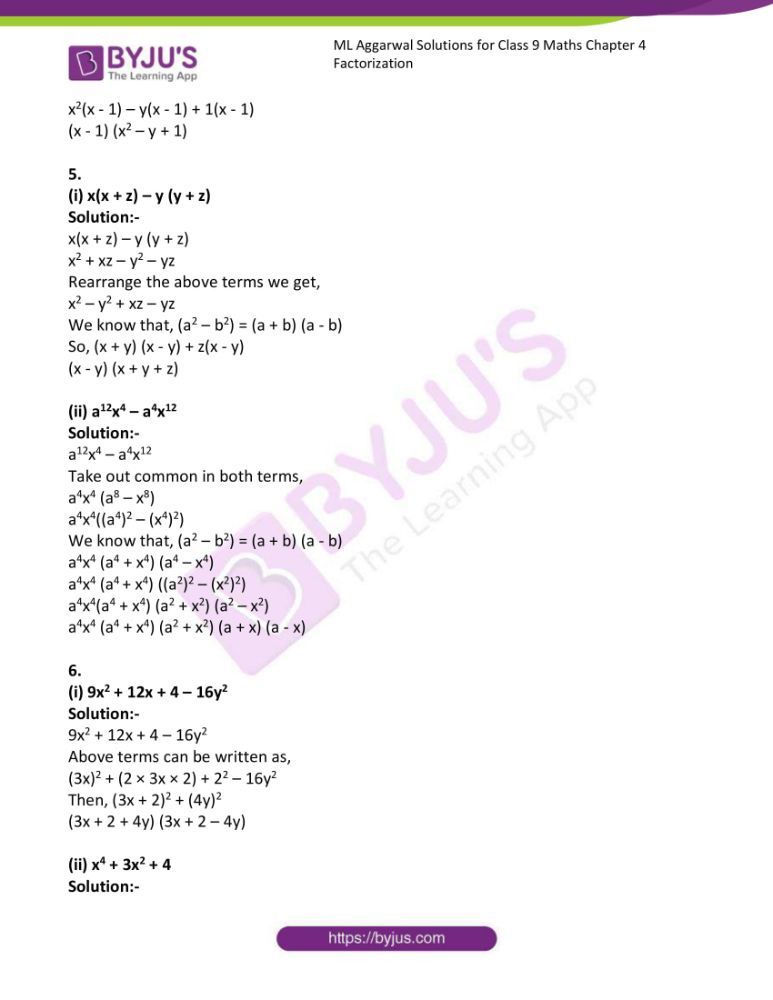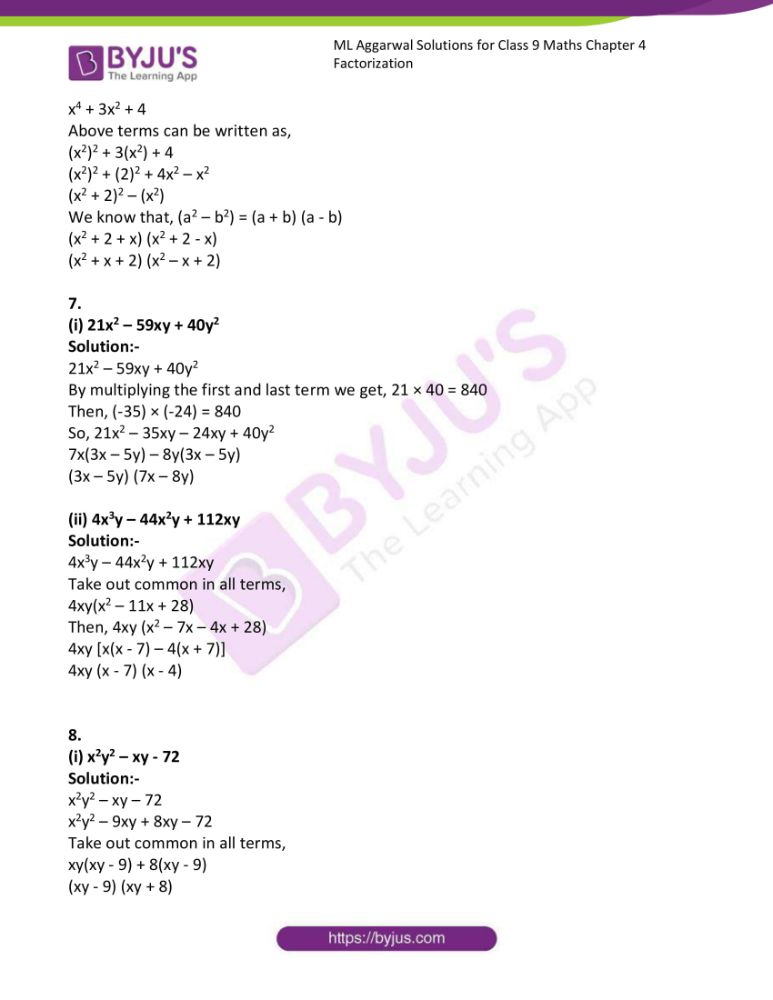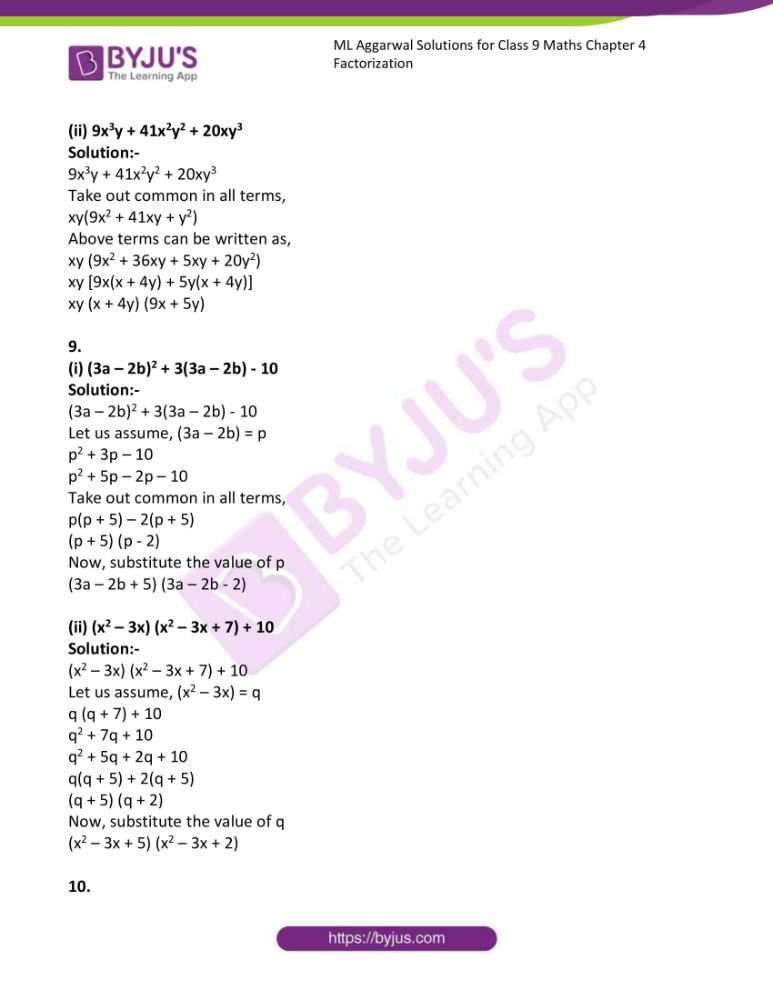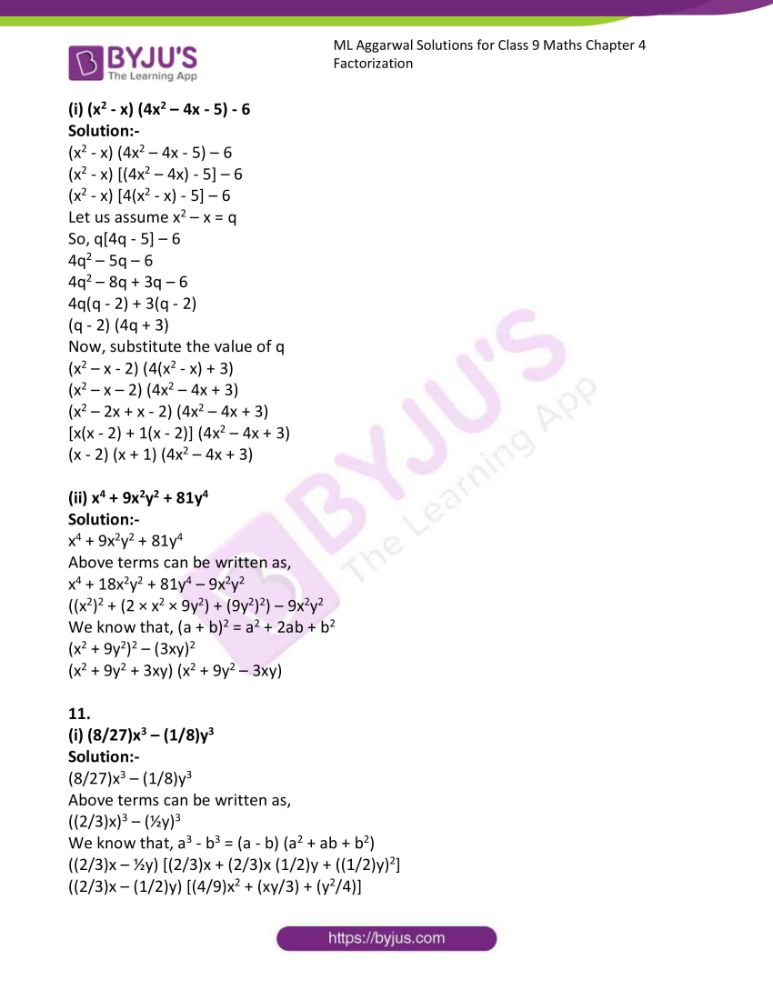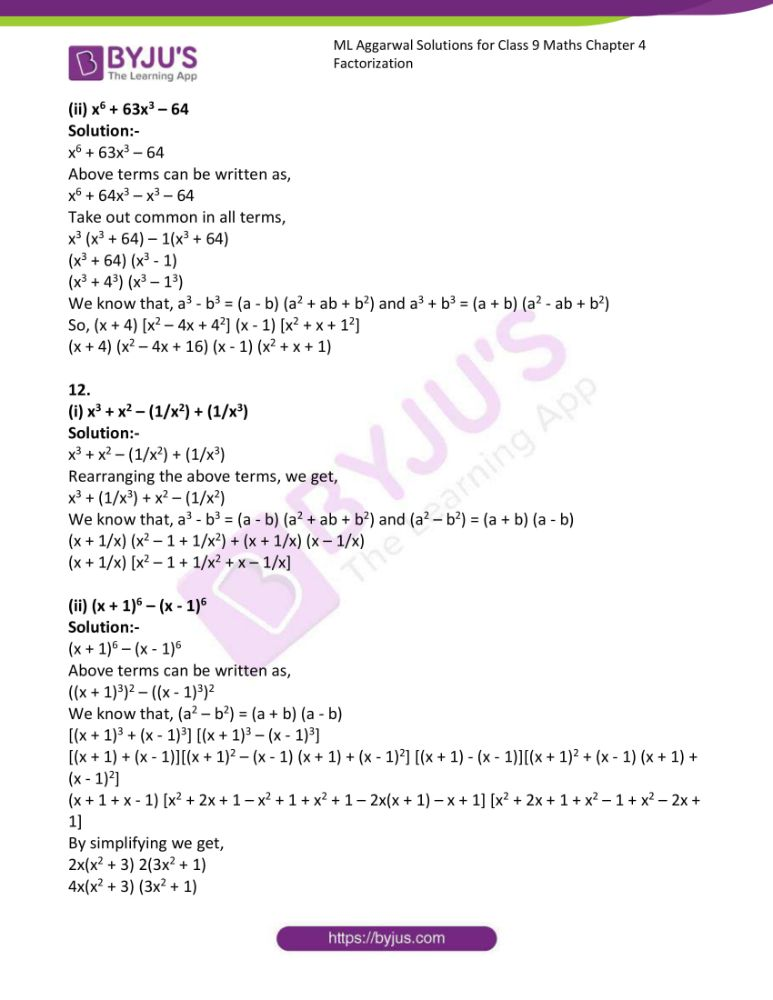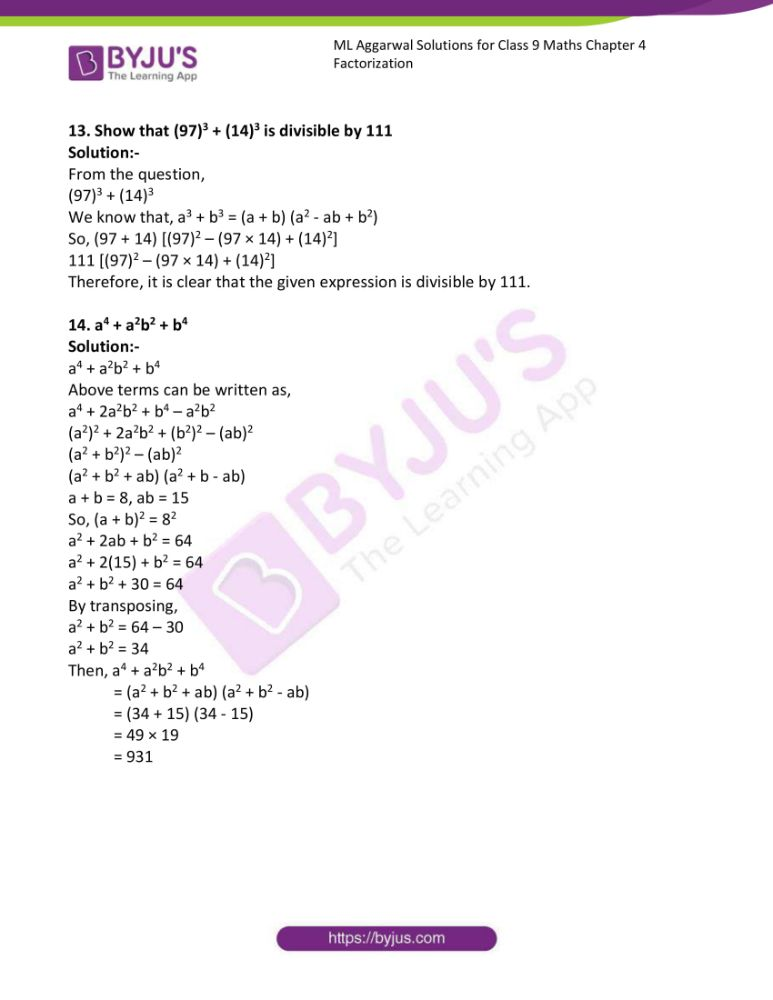### Access answers to ML Aggarwal Solutions for Class 9 Maths Chapter 4 Factorization

Exercise 4.1

1. (i) 8xy3 + 12x2y2

Solution:-

8xy3 + 12x2y2

Take out common in both terms,

Then, 4xy2 (2y + 3x)

Therefore, HCF of 8xy3 and 12x2y2 is 4xy2.

(ii) 15 ax3 – 9ax2

Solution:-

15 ax3 – 9ax2

Take out common in both terms,

Then, 3ax2 (5x – 3)

Therefore, HCF of 15 ax3 and 9ax2 is 3ax2.

2.

(i) 21py2 – 56py

Solution:-

21py2 – 56py

Take out common in both terms,

Then, 7py (3y – 8)

Therefore, HCF of 21py2 and 56py is 7py.

(ii) 4x3 – 6x2

Solution:-

4x3 – 6x2

Take out common in both terms,

Then, 2x2 (2x – 3)

Therefore, HCF of 4x3 and 6x2 is 2x2.

3.

(i) 2πr2 – 4πr

Solution:-

2πr2 – 4πr

Take out common in both terms,

Then, 2πr (r – 2)

Therefore, HCF of 2πr2 and 4πr is 2πr.

(ii) 18m + 16n

Solution:-

18m + 16n

Take out common in both terms,

Then, 2 (9m – 8n)

Therefore, HCF of 18m and 16n is 2.

4.

(i) 25abc2 – 15a2b2c

Solution:-

25abc2 – 15a2b2c

Take out common in both terms,

Then, 5abc (5c – 3ab)

Therefore, HCF of 25abc2 and 15a2b2c is 5abc.

(ii) 28p2q2r – 42pq2r2

Solution:-

28p2q2r – 42pq2r2

Take out common in both terms,

Then, 14pq2r (2p – 3r)

Therefore, HCF of 28p2q2r and 42pq2r2 is 14pq2r.

5.

(i) 8x3 – 6x2 + 10x

Solution:-

8x3 – 6x2 + 10x

Take out common in both terms,

Then, 2x(4x2 – 3x + 5)

Therefore, HCF of 8x3, 6x2 and 10x is 2x.

(ii) 14mn + 22m – 62p

Solution:-

14mn + 22m – 62p

Take out common in both terms,

Then, 2 (7mn + 11m – 31p)

Therefore, HCF of 14mn, 22m and 62p is 2.

6.

(i) 18p2q2 – 24pq2 + 30p2q

Solution:-

18p2q2 – 24pq2 + 30p2q

Take out common in both terms,

Then, 6pq (3pq – 4q + 5p)

Therefore, HCF of 18p2q2, 24pq2 and 30p2q is 6pq.

(ii) 27a3b3 – 18a2b3 + 75a3b2

Solution:-

27a3b3 – 18a2b3 + 75a3b2

Take out common in both terms,

Then, 3a2b2 (9a – 6b + 25a)

Therefore, HCF of 27a3b3, 18a2b3 and 75a3b2 is 3a2b2.

7.

(i) 15a (2p – 3q) – 10b (2p – 3q)

Solution:-

15a (2p – 3q) – 10b (2p – 3q)

Take out common in both terms,

Then, 5(2p – 3q) [3a – 2b]

Therefore, HCF of 15a (2p – 3q) and 10b (2p – 3q) is 5(2p – 3q).

(ii) 3a(x2 + y2) + 6b (x2 + y2)

Solution:-

3a(x2 + y2) + 6b (x2 + y2)

Take out common in all terms,

Then, 3(x2 + y2) (a + 2b)

Therefore, HCF of 3a(x2 + y2) and 6b (x2 + y2) is 3(x2 + y2).

8.

(i) 6(x + 2y)3 + 8(x + 2y)2

Solution:-

6(x + 2y)3 + 8(x + 2y)2

Take out common in all terms,

Then, 2(x + 2y)2 [3(x + 2y) + 4]

Therefore, HCF of 6(x + 2y)3 and 8(x + 2y)2 is 2(x + 2y)2.

(ii) 14(a – 3b)3 – 21p(a – 3b)

Solution:-

14(a – 3b)3 – 21p(a – 3b)

Take out common in all terms,

Then, 7(a – 3b) [2(a – 3b)2 – 3p]

Therefore, HCF of 14(a – 3b)3 and 21p(a – 3b) is 7(a – 3b).

9.

(i) 10a(2p + q)3 – 15b (2p + q)2 + 35 (2p + q)

Solution:-

10a(2p + q)3 – 15b (2p + q)2 + 35 (2p + q)

Take out common in all terms,

Then, 5(2p + q) [2a (2p + q)2 – 3b (2p + q) + 7]

Therefore, HCF of 10a(2p + q)3, 15b (2p + q)2 and 35 (2p + q) is 5(2p + q).

(ii) x(x2 + y2 – z2) + y(-x2 – y2 + z2) – z (x2 + y – z2)

Solution:-

x(x2 + y2 – z2) + y(-x2 – y2 + z2) – z (x2 + y – z2)

Take out common in all terms,

Then, (x2 + y2 – z2) [x – y – z]

Therefore, HCF of x(x2 + y2 – z2), y(-x2 – y2 + z2) and z (x2 + y – z2) is (x2 + y2 – z2)

Exercise 4.2

1.

(i) x2 + xy – x – y

Solution:-

x2 + xy – x – y

Take out common in all terms,

x(x + y) – 1(x + y)

(x + y) (x – 1)

(ii) y2 – yz – 5y + 5z

Solution:-

y2 – yz – 5y + 5z

Take out common in all terms,

y(y – z) – 5(y – z)

(y – z) (y – 5)

2.

(i) 5xy + 7y – 5y2 – 7x

Solution:-

5xy – 7x – 5y2 + 7y

Take out common in all terms,

x(5y – 7) – y(5y – 7)

(5y – 7) (x – y)

(ii) 5p2 – 8pq – 10p + 16q

Solution:-

5p2 – 8pq – 10p + 16q

Take out common in all terms,

p(5p – 8q) – 2(5p – 8q)

(5p – 8q) (p – 2)

3.

(i) a2b – ab2 + 3a – 3b

Solution:-

a2b – ab2 + 3a – 3b

Take out common in all terms,

ab(a – b) + 3(a – b)

(a – b) (ab + 3)

(ii) x3 – 3x2 + x – 3

Solution:-

x3 – 3x2 + x – 3

Take out common in all terms,

x2 (x – 3) + 1(x – 3)

(x – 3) (x2 + 1)

4.

(i) 6xy2 – 3xy – 10y + 5

Solution:-

6xy2 – 3xy – 10y + 5

Take out common in all terms,

3xy(2y – 1) – 5(2y – 1)

(2y – 1) (3xy – 5)

(ii) 3ax – 6ay – 8by + 4bx

Solution:-

3ax – 6ay – 8by + 4bx

Take out common in all terms,

3a(x – 2y) + 4b (x – 2y)

(x – 2y) (3a + 4b)

5.

(i) 1 – a – b + ab

Solution:-

1 – a – b + ab

Take out common in all terms,

1(1 – a) – b(1 – a)

(1 – a) (1 – b)

(ii) a(a – 2b – c) + 2bc

Solution:-

a(a – 2b – c) + 2bc

Above question can be written as,

a2 – 2ab – ac + 2bc

Take out common in all terms,

a(a – 2b) – c(a + 2b)

(a – 2b) (a – c)

6.

(i) x2 + xy (1 + y) + y3

Solution:-

x2 + xy (1 + y) + y3

Above question can be written as,

x2 + xy + xy2 + y3

Take out common in all terms,

x(x + y) + y2(x + y)

(x + y) (x + y2)

(ii) y2 – xy (1 – x) – x3

Solution:-

y2 – xy (1 – x) – x3

Above question can be written as,

y2 – xy + x2y – x3

Take out common in all terms,

y(y – x) + x2 (y – x)

(y – x) (y + x2)

7.

(i) ab2 + (a – 1)b – 1

Solution:-

ab2 + (a – 1)b – 1

Above question can be written as,

ab2 + ab – b – 1

Take out common in all terms,

ab(b + 1) – 1(b + 1)

(b + 1) (ab – 1)

(ii) 2a – 4b – xa + 2bx

Solution:-

2a – 4b – xa + 2bx

Take out common in all terms,

2(a – 2b) – x(a – 2b)

(a – 2b) (2 – x)

8.

(i) 5ph – 10qk + 2rph – 4qrk

Solution:-

5ph – 10qk + 2rph – 4qrk

Re-arranging the given question we get,

5ph + 2rph – 10qk – 4qrk

Take out common in all terms,

ph(5 + 2r) – 2qk(5 + 2r)

(5 + 2r) (ph – 2qk)

(ii) x2 – x(a + 2b) + 2ab

Solution:-

x2 – x(a + 2b) + 2ab

Above question can be written as,

x2 – xa – 2xb + 2ab

Take out common in all terms,

x(x – a) – 2b(x – a)

(x – a) (x – 2b)

9.

(i) ab(x2 + y2) – xy(a2 + b2)

Solution:-

ab(x2 + y2) – xy(a2 + b2)

Above question can be written as,

abx2 + aby2 – xya2 – xyb2

Re-arranging the above we get,

abx2 – xyb2 + aby2 – xya2

Take out common in all terms,

bx(ax – by) + ay(by – ax)

bx(ax – by) – ay (ax – by)

(ax – by) (bx – ay)

(ii) (ax + by)2 + (bx – ay)2

Solution:-

By expanding the give question, we get,

(ax)2 + (by)2 + 2axby + (bx)2 + (ay)2 – 2bxay

a2x2 + b2y2 + b2x2 + a2y2

Re-arranging the above we get,

a2x2 + a2y2 + b2y2 + b2x2

Take out common in all terms,

a2 (x2 + y2) + b2 (x2 + y2)

(x2 + y2) (a2 + b2)

10.

(i) a3 + ab(1 – 2a) – 2b2

Solution:-

a3 + ab(1 – 2a) – 2b2

Above question can be written as,

a3 + ab – 2a2b – 2b2

Re-arranging the above we get,

a3 – 2a2b + ab – 2b2

Take out common in all terms,

a2(a – 2b) + b(a – 2b)

(a – 2b) (a2 + b)

(ii) 3x2y – 3xy + 12x – 12

Solution:-

3x2y – 3xy + 12x – 12

Take out common in all terms,

3xy(x – 1) + 12(x – 1)

(x – 1) (3xy + 12)

11. a2b + ab2 –abc – b2c + axy + bxy

Solution:-

a2b + ab2 –abc – b2c + axy + bxy

Re-arranging the above we get,

a2b – abc + axy + ab2 – b2c + bxy

Take out common in all terms,

a(ab – bc + xy) + b(ab – bc + xy)

(a + b) (ab – bc + xy)

12. ax2 – bx2 + ay2 – by2 + az2 – bz2

Solution:-

ax2 – bx2 + ay2 – by2 + az2 – bz2

Re-arranging the above we get,

ax2 + ay2 + az2 – bx2 – by2 – bz2

Take out common in all terms,

a(x2 + y2 + z2) – b(x2 + y2 + z2)

(x2 + y2 + z2) (a – b)

13. x – 1 – (x – 1)2 + ax – a

Solution:-

x – 1 – (x – 1)2 + ax – a

By expanding the above we get,

X – 1 – (x2 + 1 – 2x) + ax – a

x – 1 – x2 -1 + 2x + ax – a

2x – x2 + ax – 2 + x – a

Take out common in all terms,

x(2 – x + a) – 1(2 – x + a)

(2 – x + a) (x – 1)

Exercise 4.3

1. 4x2 – 25y2

Solution:-

We know that, a2 – b2 = (a + b) (a – b)

So, (2x)2 – (5y)2

Then, (2x + y) (2x – 5y)

(ii) 9x2 – 1

Solution:-

We know that, a2 – b2 = (a + b) (a – b)

So, (3x)2 – 12

Then, (3x + 1) (3x – 1)

2.

(i) 150 – 6a2

Solution:-

150 – 6a2

Take out common in all terms,

6(25 – a2)

6(52 – a2)

We know that, a2 – b2 = (a + b) (a – b)

So, 6(5 + a) (5 – a)

(ii) 32x2 – 18y2

Solution:-

32x2 – 18y2

Take out common in all terms,

2(16x2 – 9y2)

2((4x)2 – (3y)2)

We know that, a2 – b2 = (a + b) (a – b)

2(4x + 3y) (4x – 3y)

3.

(ii) (x – y)2 – 9

Solution:-

(x – y)2 – 9

(x – y)2 – 32

We know that, a2 – b2 = (a + b) (a – b)

(x – y + 3) (x – y – 3)

(ii) 9(x + y)2 – x2

Solution:-

9[(x + y)2 – x2]

We know that, a2 – b2 = (a + b) (a – b)

9[(x + y + x) (x + y – x)]

So, 9(2x + y) y

9y(2x + y)

4.

(i) 20x2 – 45y2

Solution:-

20x2 – 45y2

Take out common in all terms,

5(4x2 – 9y2)

5((2x)2 – (3y)2)

We know that, a2 – b2 = (a + b) (a – b)

5(2x + 3y) (2x – 3y)

(ii) 9x2 – 4(y + 2x)2

Solution:-

9x2 – 4(y + 2x)2

Above question can be written as,

(3x)2 – [2(y + 2x)]2

(3x)2 – (2y + 4x)2

We know that, a2 – b2 = (a + b) (a – b)

(3x + 2y + 4x) (3x – 2y – 4x)

(7x + 2y) (-x – 2y)

5.

(i) 2(x – 2y)2 – 50y2

Solution:-

2(x – 2y)2 – 50y2

Take out common in all terms,

2[(x – 2y)2 – 25y2]

2[(x – 2y)2 – (5y)2]

We know that, a2 – b2 = (a + b) (a – b)

2[(x – 2y + 5y) (x – 2y – 5y)]

2[(x + 3y) (x – 7y)]

2(x + 3y) (x – 7y)

(ii) 32 – 2(x – 4)2

Solution:-

32 – 2(x – 4)2

Take out common in all terms,

2[16 – (x – 4)2]

2[42– (x – 4)2]

We know that, a2 – b2 = (a + b) (a – b)

2[(4 + x – 4) (4 – x + 4)]

2[(x) (8 – x)]

2x (8 – x)

6.

(i) 108a2 – 3(b – c)2

Solution:-

108a2 – 3(b – c)2

Take out common in all terms,

3[36a2 – (b – c)2]

3[(6a)2 – (b – c)2]

We know that, a2 – b2 = (a + b) (a – b)

3[(6a + b – c) (6a – b + c)]

(ii) πa5 – π3ab2

Solution:-

πa5 – π3ab2

Take out common in all terms,

πa(a4 – π2b2)

πa((a2)2 – (πb)2)

We know that, a2 – b2 = (a + b) (a – b)

πa(a2 + πb) (a2 – πb)

7.

(i) 50x2 – 2(x – 2)2

Solution:-

50x2 – 2(x – 2)2

Take out common in all terms,

2[25x2 – (x – 2)2]

2[(5x)2 – (x – 2)2]

We know that, a2 – b2 = (a + b) (a – b)

2[(5x + x – 2) (5x – x + 2)]

2[(6x – 2) (4x + 2)]

2(6x – 2) (4x + 2)

(ii) (x – 2)(x + 2) + 3

Solution:-

We know that, a2 – b2 = (a + b) (a – b)

(x2 – 22) + 3

X2 – 4 + 3

X2 – 1

Then,

(x + 1) (x – 1)

8.

(i) x – 2y – x2 + 4y2

Solution:-

x – 2y – x2 + 4y2

x – 2y – (x2 + (2y)2)

We know that, a2 – b2 = (a + b) (a – b)

x – 2y – [(x + 2y) (x – 2y)]

Take out common in all terms,

(x – 2y) (1 – (x + 2y))

(x – 2y) (1 – x – 2y)

(ii) 4a2 – b2 + 2a + b

Solution:-

4a2 – b2 + 2a + b

(2a)2 – b2 + 2a + b

We know that, a2 – b2 = (a + b) (a – b)

((2a + b) (2a – b)) + 1(2a + b)

Take out common in all terms,

(2a + b) (2a – b + 1)

9.

(i) a(a – 2) – b(b – 2)

Solution:-

a(a – 2) – b(b – 2)

Above question can be written as,

a2 – 2a – b2 – 2b

Rearranging the above terms, we get,

a2 – b2 – 2a – 2b

We know that, a2 – b2 = (a + b) (a – b)

[(a + b)(a – b)] – 2(a – b)

Take out common in all terms,

(a – b) (a + b – 2)

(ii) a(a – 1) – b(b – 1)

Solution:-

a(a – 1) – b(b – 1)

Above question can be written as,

a2 – a – b2 + b

Rearranging the above terms, we get,

a2 – b2 – a + b

We know that, a2 – b2 = (a + b) (a – b)

[(a + b) (a – b)] – 1 (a – b)

Take out common in all terms,

(a – b) (a + b – 1)

10.

(i) 9 – x2 + 2xy – y2

Solution:-

9 – x2 + 2xy – y2

9 – x2 + 2xy – y2

Above terms can be written as,

9 – x2 + xy + xy – y2

Now,

9 – x2 + xy + 3x – 3x + 3y – 3y + xy – y2

Rearranging the above terms, we get,

9 – 3x + 3y + 3x – x2 + xy + xy – 3y – y2

Take out common in all terms,

3(3 – x + y) + x(3 – x + y) + y (-3 – y + x)

3(3 – x + y) + x(3 – x + y) – y(3 – x + y)

(3 – x + y) (3 + x – y)

(ii) 9x4 – (x2 + 2x + 1)

Solution:-

9x4 – (x2 + 2x + 1)

Above terms can be written as,

(3x2)2 – (x + 1)2 … [because (a + b)2 = a2 + 2ab + b2]

We know that, a2 – b2 = (a + b) (a – b)

So, (3x2 + x + 1) (3x2 – x – 1)

11.

(i) 9x4 – x2 – 12x – 36

Solution:-

9x4 – x2 – 12x – 36

Above terms can be written as,

9x4 – (x2 + 12x + 36)

We know that, (a + b)2 = a2 + 2ab + b2

(3x2)2 – (x2 + (2 × 6 × x) + 62)

So, (3x2)2 – (x + 6)2

We know that, a2 – b2 = (a + b) (a – b)

(3x2 + x + 6) (3x2 – x – 6)

(ii) x3 – 5x2 – x + 5

Solution:-

x3 – 5x2 – x + 5

Take out common in all terms,

x2(x – 5) – 1(x – 5)

(x – 5) (x2 – 1)

(x – 5) (x2 – 12)

We know that, a2 – b2 = (a + b) (a – b)

(x – 5) (x + 1) (x – 1)

12.

(i) a4 – b4 + 2b2 – 1

Solution:-

a4 – b4 + 2b2 – 1

Above terms can be written as,

a4 – (b4 – 2b2 + 1)

We know that, (a – b)2 = a2 – 2ab + b2

a4 – ((b2)2) – (2 × b2 × 1) + 12)

(a2)2 – (b2 – 1)2

We know that, a2 – b2 = (a + b) (a – b)

(a2 + b2 – 1) (a2 – b2 + 1)

(ii) x3 – 25x

Solution:-

x3 – 25x

Take out common in all terms,

x(x2 – 25)

Above terms can be written as,

x(x2 – 52)

We know that, a2 – b2 = (a + b) (a – b)

x(x + 5) (x – 5)

13.

(i) 2x4 – 32

Solution:-

2x4 – 32

Take out common in all terms,

2(x4 – 16)

Above terms can be written as,

2((x2)2 – 42)

We know that, a2 – b2 = (a + b) (a – b)

2(x2 + 4) (x2 – 4)

2(x2 + 4) (x2 – 22)

2(x2 + 4) (x + 2) (x – 2)

(ii) a2(b + c) – (b + c)3

Solution:-

a2(b + c) – (b + c)3

Take out common in all terms,

(b + c) (a2 – (b + c)2)

We know that, a2 – b2 = (a + b) (a – b)

(b + c) (a + b + c) (a – b – c)

14.

(i) (a + b)3 – a – b

Solution:-

(a + b)3 – a – b

Above terms can be written as,

(a + b)3 – (a + b)

Take out common in all terms,

(a + b) [(a + b)2 – 1]

(a + b) [(a + b)2 – 12]

We know that, a2 – b2 = (a + b) (a – b)

(a + b) (a + b + 1) (a + b – 1)

(ii) x2 – 2xy + y2 – a2 – 2ab – b2

Solution:-

x2 – 2xy + y2 – a2 – 2ab – b2

Above terms can be written as,

(x2 – 2xy + y2) – (a2 + 2ab + b2)

We know that, (a + b)2 = a2 + 2ab + b2 and (a – b)2 = a2 – 2ab + b2

(x2 – (2 × x × y) + y2) – (a2 + (2 × a × b) + b2)

(x – y)2 – (a + b)2

We know that, a2 – b2 = (a + b) (a – b)

[(x – y) + (a + b)] [(x – y) – (a + b)]

(x – y + a + b) (x – y – a – b)

15.

(i) (a2 – b2) (c2 – d2) – 4abcd

Solution:-

(a2 – b2) (c2 – d2) – 4abcd

a2(c2 – d2) – b2 (c2 – d2) – 4abcd

a2c2 – a2d2 – b2c2 + b2d2 – 4abcd

a2c2 + b2d2 – a2d2 – b2c2 – 2abcd – 2abcd

Rearranging the above terms, we get,

a2c2 + b2d2 – 2abcd – a2d2 – b2c2 – 2abcd

We know that, (a + b)2 = a2 + 2ab + b2 and (a – b)2 = a2 – 2ab + b2

(ac – bd)2 – (ad – bc)2

(ac – bd + ad – bc) (ac – bd – ad + bc)

(ii) 4x2 – y2 – 3xy + 2x – 2y

Solution:-

4x2 – y2 – 3xy + 2x – 2y

Above terms can be written as,

x2 + 3x2 – y2 – 3xy + 2x – 2y

Rearranging the above terms, we get,

(x2 – y2) + (3x2 – 3xy) + (2x – 2y)

We know that, a2 – b2 = (a + b) (a – b) and take out common terms,

(x + y) (x – y) + 3x(x – y) + 2(x – y)

(x – y) [(x + y) + 3x + 2]

(x – y) (x + y + 3x + 2)

(x – y) (4x + y + 2)

16.

(i) x2 + 1/x2 – 11

Solution:-

x2 + 1/x2 – 11

Above terms can be written as,

x2 + (1/x2) – 2 – 9

Then, (x2 + (1/x2) – 2) – 32

We know that, (a – b)2 = a2 – 2ab + b2,

(x2 – (2 × x2 × (1/x2)) + (1/x)2)

(x – 1/x)2 – 32

We know that, a2 – b2 = (a + b) (a – b)

(x – 1/x + 3) (x – 1/x – 3)

(ii) x4 + 5x2 + 9

Solution:-

x4 + 5x2 + 9

x4 + 6x2 – x2 + 9

(x4 + 6x2 + 9) – x2

((x2)2 + (2 × x2 × 3) + 32)

We know that, (a + b)2 = a2 + 2ab + b2,

((x2)2 + (2 × x2 × 3) + 32)

So, (x2 + 3)2 – x2

We know that, a2 – b2 = (a + b) (a – b)

(x2 + 3 + x) (x2 + 3 – x)

17.

(i) a4 + b4 – 7a2b2

Solution:-

a4 + b4 – 7a2b2

Above terms can be written as,

a4 + b4 + 2a2b2 – 9a2b2

We know that, (a + b)2 = a2 + 2ab + b2,

[(a2)2 + (b2)2 + (2 × a2 × b2)] – (3ab)2

(a2 + b2)2 – (3ab)2

We know that, a2 – b2 = (a + b) (a – b)

(a2 + b2 + 3ab) (a2 + b2 – 3ab)

(ii) x4 – 14x2 + 1

Solution:-

x4 – 14x2 + 1

Above terms can be written as,

x4 + 2x2 + 1 – 16x2

We know that, (a + b)2 = a2 + 2ab + b2,

So, [(x2)2 + (2 × x2 × 1) + 12] – 16x2

(x2 + 1)2 – (4x)2

We know that, a2 – b2 = (a + b) (a – b)

(x2 + 1 + 4x) (x2 + 1 – 4x)

18.

(i) (x2 – 5x + 7) (x2 + 5x + 7)

Solution:-

(x2 – 5x + 7) (x2 + 5x + 7)

Rearranging the above terms, we get,

((x2 + 7) – 5x) ((x2 + 7) + 5x)

As, we know that, a2 – b2 = (a + b) (a – b)

So, (x2 + 7)2 – (5x)2

(x2 + 7)2 -25x2

(ii) (x2 – 5x + 7) (x2 – 5x – 7)

Solution:-

(x2 – 5x + 7) (x2 – 5x – 7)

[(x2 – 5x) + 7) ((x2 – 5x) – 7)

As, we know that, a2 – b2 = (a + b) (a – b)

(x2 – 5x)2 – 72

(x2 – 5x)2 – 49

(iii) (x2 + 5x – 7) (x2 – 5x + 7)

Solution:-

(x2 + 5x – 7) (x2 – 5x + 7)

[x2 + (5x – 7)] [x2 – (5x – 7)]

As, we know that, a2 – b2 = (a + b) (a – b)

x2 – (5x – 7)2

We know that, (a – b)2 = a2 – 2ab + b2,

X2 – [(5x)2 – (2 × 5x × 7) + 72]

X2 – (25x2 – 70x + 49)

X2 – 25x2 + 70x – 49

-24x2 + 70x – 49

19.

(i) x – 1/x = 5/6

Solution:-

x – 1/x = 5/6

Now, squaring on both side we get,

X2 + (1/x2) – 2 = 25/36

Transposing – 2 from LHS to RHS then it becomes 2.

x2 + (1/x2) = (25/36) + 2

x2 + (1/x2) = (25 + 72)/36

x2 + 1/x2 = 97/36

(ii) (x + 1/x)2 = (x2 + (1/x2) + 2)

Solution:-

(x + 1/x)2 = (x2 + (1/x2) + 2)

From (i), x2 + 1/x2 = 97/36

So, (x + 1/x)2 = (97/36) + 2

(x + 1/x)2 = (97 + 72)/36

(x + 1/x)2 = 169/36

x + (1/x) = √(169/36)

x + (1/x) = 13/6

(iii) (x + 1/x)3 = x3 + (1/x3) + 3(x + (1/x))

Solution:-

(x + 1/x)3 = x3 + (1/x3) + 3(x + (1/x))

From (ii), x + (1/x) = 13/6

(13/6)3 = x3 + (1/x3) + 3(13/6)

2197/216 = x3 + (1/x3) + 39/6

x3 + (1/x3) = (2197/216) – (39/6)

x3 + (1/x3) = (2197 – 1404)/216

x3 + (1/x3) = 793/216

(iv) x4 – 1/x4

Solution:-

x4 – 1/x4 = (x2 + 1/x2) (x2 – 1/x2)

= (x2 + 1/x2) (x + 1/x) (x – 1/x)

From (i), (ii) and (iii) substitute the values we get,,

= (97/36) × (13/6) × (5/6)

= 6305/1296

20. If x + 1/x = √3, find the values of

(i) x3 + 1/x3

Solution:-

From question, x + 1/x = √3

x + 1/x = √3

Cubing on both LHS and RHS we get,

(x + 1/x)3 = (√3)3

X3 + (1/x3) + 3(x + (1/x)) = 3√3

x3 + 1/x3 + 3(√3) = 3√3

By transposing we get,

x3 + 1/x3 + 3√3 – 3√3 = 0

x3 + 1/x3 = 0

(ii) x6 – 1/x6

Solution:-

x6 – 1/x6

Above terms can be written as,

= (x3)2 – (1/x3)2

We know that, (a – b)2 = a2 – 2ab + b2,

(x3 + (1/x3)) (x3 – (1/x3))

From (i) x3 + 1/x3 = 0

So, 0 × (x3 – 1/x3)

Exercise 4.4

1.

(i) x2 + 5x + 6

Solution:-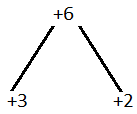x2 + 5x + 6

x2 + 3x + 2x + 6

Take out common in all terms we get,

x(x + 3) + 2 (x + 3)

(x + 3) (x + 2)

(ii) x2 – 8x + 7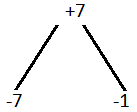Solution:-

x2 – 8x + 7

x2 – 7x – x + 7

Take out common in all terms we get,

x(x – 7) – 1(x – 7)

(x – 7) (x – 1)

2.

(i) x2 + 6x – 7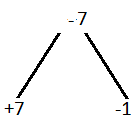Solution:-

x2 + 6x – 7

x2 + 7x – x – 7

Take out common in all terms we get,

x(x + 7) – 1(x + 7)

(x + 7) (x – 1)

(ii) y2 + 7y – 18

Solution:-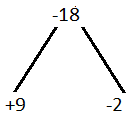y2 + 7y – 18

y2 + 9y – 2y – 18

Take out common in all terms we get,

y(y + 9) – 2(y + 9)

(y + 9) (y – 2)

3.

(i) y2 – 7y – 18

Solution:-

y2 – 7y – 18

y2 + 2y – 9y – 18

Take out common in all terms we get,

y(y + 2) – 9(y + 2)

(y + 2) (y – 9)

(ii) a2 – 3a – 54

Solution:-

a2 – 3a – 54

a2 + 6a – 9a – 54

Take out common in all terms we get,

a(a + 6) – 9(a + 6)

So, (a + 6) (a – 9)

4.

(i) 2x2 – 7x + 6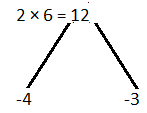Solution:-

2x2 – 7x + 6

2x2 – 4x – 3x + 6

Take out common in all terms we get,

2x(x – 2) – 3(x – 2)

(x – 2) (2x – 3)

(ii) 6x2 + 13x – 5

Solution:-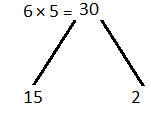6x2 + 13x – 5

6x2 + 15x – 2x – 5

Take out common in all terms we get,

3x(2x + 5) – 1(2x + 5)

(2x + 5) (3x – 1)

5.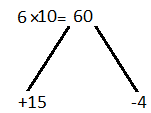(i) 6x2 + 11x – 10

Solution:-

6x2 + 11x – 10

6x2 + 15x – 4x – 10

Take out common in all terms we get,

3x(2x + 5) – 2(2x + 5)

(2x + 5) (3x – 2)

(ii) 6x2 – 7x – 3

Solution:-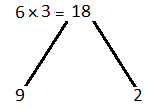6x2 – 7x – 3

6x2 – 9x + 2x – 3

Take out common in all terms we get,

3x(2x – 3) + 1(2x – 3)

(2x – 3) (3x + 1)

6.

(i) 2x2 – x – 6

Solution:-

2x2 – x – 6

2x2 – 4x + 3x – 6

Take out common in all terms we get,

2x(x – 2) + 3(x – 2)

(x – 2) (2x + 3)

(ii) 1 – 18y – 63y2

Solution:-

1 – 18y – 63y2

1 – 21y + 3y – 63y2

Take out common in all terms we get,

1(1 – 21y) + 3y(1 – 21y)

(1 – 21y) (1 + 3y)

7.

(i) 2y2 + y – 45

Solution:-

2y2 + y – 45

2y2 + 10y – 9y – 45

Take out common in all terms we get,

2y (y + 5) – 9(y + 5)

(y + 5) (2y – 9)

(ii) 5 – 4x – 12x2

Solution:-

5 – 4x – 12x2

5 – 10x + 6x – 12x2

Take out common in all terms we get,

5(1 – 2x) + 6x(1 – 2x)

(1 – 2x) (5 + 6x)

8.

(i) x(12x + 7) – 10

Solution:-

x(12x + 7) – 10

Above terms can be written as,

12x2 + 7x – 10

12x2 + 15x – 8x – 10

Take out common in all terms we get,

3x(4x + 5) – 2(4x + 5)

(4x + 5) (3x – 2)

(ii) (4 – x)2 – 2x

Solution:-

(4 – x)2 – 2x

We know that, (a – b)2 = a2 – 2ab + b2

So, (42 – (2 × 4 × x) + x2) – 2x

16 – 8x + x2 – 2x

x2 – 10x + 16

x2 – 8x – 2x + 16

Take out common in all terms we get,

x(x – 8) – 2(x – 8)

(x – 8) (x -2)

9.

(i) 60x2 – 70x – 30

Solution:-

60x2 – 70x – 30

Take out common in all terms we get,

10(6x2 – 7x – 3)

10(6x2 – 9x + 2x – 3)

Again, take out common in all terms we get,

10(3x(2x – 3) + 1(2x – 3))

10(2x – 3) (3x + 1)

(ii) x2 – 6xy – 7y2

Solution:-

x2 – 6xy – 7y2

x2 – 7xy + xy – 7y2

Take out common in all terms we get,

x(x – 7y) + y(x – 7y)

(x – 7y) (x + y)

10.

(i) 2x2 + 13xy – 24y2

Solution:-

2x2 + 13xy – 24y2

2x2 + 16xy – 3xy – 24y2

Take out common in all terms we get,

2x(x + 8y) – 3y(x + 8y)

(x + 8y) (2x – 3y)

(ii) 6x2 – 5xy – 6y2

Solution:-

6x2 – 5xy – 6y2

6x2 – 9xy + 4xy – 6y2

Take out common in all terms we get,

3x(2x – 3y) + 2y (2x – 3y)

(2x – 3y) (3x + 2y)

11.

(i) 5x2 + 17xy – 12y2

Solution:-

5x2 + 17xy – 12y2

5x2 + 20xy – 3xy – 12y2

Take out common in all terms we get,

5x(x + 4y) – 3y(x + 4y)

(x + 4y) (5x – 3y)

(ii) x2y2 – 8xy – 48

Solution:-

x2y2 – 8xy – 48

x2y2 – 12xy + 4xy – 48

Take out common in all terms we get,

xy(xy – 12) + 4(xy – 12)

(xy – 12) (xy + 4)

12.

(i) 2a2b2 – 7ab – 30

Solution:-

2a2b2 – 7ab – 30

2a2b2 – 12ab + 5ab – 30

Take out common in all terms we get,

2ab(ab – 6) + 5 (ab – 6)

(ab – 6) (2ab + 5)

(ii) a(2a – b) – b2

Solution:-

a(2a – b) – b2

Above terms can be written as,

2a2 – ab – b2

2a2 – 2ab + ab – b2

Take out common in all terms we get,

2a(a – b) + b(a – b)

(a – b) (2a + b)

13.

(i) (x – y)2 – 6(x – y) + 5

Solution:-

(x – y)2 – 6(x – y) + 5

Above terms can be written as,

(x – y)2 – 5(x – y) – (x – y) + 5

(x – y) (x – y – 5) – 1(x – y – 5)

Then,

(x – y – 5) (x – y – 1)

(ii) (2x – y)2 – 11(2x – y) + 28

Solution:-

(2x – y)2 – 11(2x – y) + 28

Above terms can be written as,

(2x – y)2 – 7(2x – y) – 4(2x – y) + 28

(2x – y) (2x – y – 7) – 4(2x – y – 7)

(2x – y – 7) (2x – y – 4)

14.

(i) 4(a – 1)2 – 4(a – 1) – 3

Solution:-

4(a – 1)2 – 4(a – 1) – 3

Above terms can be written as,

4(a – 1)2 – 6(a – 1) + 2(a – 1) – 3

Take out common in all terms we get,

2(a – 1) [2(a – 1) – 3] + 1[2(a – 1) – 3]

(2(a – 1) – 3) (2(a – 1) + 1)

(2a – 2 – 3) (2a – 2 + 1)

(2a – 5) (2a – 1)

(ii) 1 – 2a – 2b – 3(a + b)2

Solution:-

1 – 2a – 2b – 3(a + b)2

Above terms can be written as,

1 – 2(a + b) – 3(a + b)2

1 – 3(a + b) + (a + b) – 3(a + b)2

Take out common in all terms we get,

1(1 – 3(a + b)) + (a + b) (1 – (a + b))

(1 – 3(a + b)) (1 + (a + b))

(1 – 3a + 3b) (1 + a + b)

15.

(i) 3 – 5a – 5b – 12(a + b)2

Solution:-

3 – 5a – 5b – 12(a + b)2

Above terms can be written as,

3 – 5(a + b) – 12(a + b)2

3 – 9(a + b) + 4(a + b) – 12(a + b)2

Take out common in all terms we get,

3(1 – 3(a + b)) + 4(a + b) (1 – 3(a + b))

(1 – 3(a + b)) (3 + 4(a + b))

(1 – 3a – 3b) (3 + 4a + 4b)

(ii) a4 – 11a2 + 10

Solution:-

a4 – 11a2 + 10

Above terms can be written as,

a4 – 10a2 – a2 + 10

Take out common in all terms we get,

a2 (a2 – 10) – 1(a2 – 10)

(a2 – 10) (a2 – 1)

16.

(i) (x + 4)2 – 5xy -20y – 6y2

Solution:-

(x + 4)2 – 5xy -20y – 6y2

Above terms can be written as,

(x + 4)2 – 5y(x + 4) – 6y2

(x + 4)2 – 6y(x + 4) + y(x + 4) – 6y2

Take out common in all terms we get,

(x + 4) (x + 4 – 6y) + y(x + 4 – 6y)

(x – 6y + 4) (x + 4 + y)

(ii) (x2 – 2x2) – 23(x2 – 2x) + 120

Solution:-

(x2 – 2x2) – 23(x2 – 2x) + 120

Above terms can be written as,

(x2 – 2x)2 – 15(x2 – 2x) – 8(x2 – 2x) + 120

Take out common in all terms we get,

(x2 – 2x) (x2 – 2x – 15) – 8(x2 – 2x – 15)

(x2 – 2x – 15) (x2 – 2x – 8)

17. 4(2a – 3)2 – 3(2a – 3) (a – 1) – 7 (a – 1)2

Solution:-

4(2a – 3)2 – 3(2a – 3) (a – 1) – 7 (a – 1)2

Let us assume, 2a – 3 = p and a – 1 = q

So, 4p2 – 3pq – 7q2

Then, 4p2 – 7pq + 4pq – 7q2

Take out common in all terms we get,

P(4p – 7q) + q(4p – 7q)

(4p – 7q) (p + q)

Now, substitute the value of p and q we get,

(4(2a – 3) – 7(a – 1)) (2a – 3 + a – 1)

(8a – 12 – 7a + 7) (3a – 4)

(a – 5) (3a – 4)

18. (2x2 + 5x) (2x2 + 5x – 19) + 84

Solution:-

(2x2 + 5x) (2x2 + 5x – 19) + 84

Let us assume, 2x2 + 5x = p

So, (p) (p – 19) + 84

p2 – 19p + 84

p2 – 12p – 7p + 84

p(p – 12) – 7(p – 12)

(p – 12) (p – 7)

Now, substitute the value of p we get,

(2x2 + 5x – 12) (2x2 + 5x – 7)

Exercise 4.5

1.

(i) 8x3 + y3

Solution:-

8x3 + y3

Above terms can be written as,

(2x)3 + y3

We know that, a3 + b3 = (a + b) (a2 – ab + b2)

Where, a = 2x, b = y

Then, (2x)3 + y3 = (2x + y) ((2x)2 – (2x × y) + y2)

= (2x + y) (4x2 – 2xy + y2)

(ii) 64x3 – 125y3

Solution:-

64x3 – 125y3

Above terms can be written as,

(4x)3 – (5y)3

We know that, a3 – b3 = (a – b) (a2 + ab + b2)

Where, a = 4x, b = 5y

Then, (4x)3 – (5y)3 = (4x – 5y) ((4x)2 + (4x × 5y) + 5y2)

= (4x – 5y) (16x2 + 2oxy + 25y2)

2.

(i) 64x3 + 1

Solution:-

64x3 + 1

Above terms can be written as,

(4x)3 + 13

We know that, a3 + b3 = (a + b) (a2 – ab + b2)

Where, a = 4x, b = 1

Then, (4x)3 + 13 = (4x + 1) ((4x)2 – (4x × 1) + 12)

= (4x + 1) (16x2 – 4x + 1)

(ii) 7a3 + 56b3

Solution:-

7a3 + 56b3

Take out common in all terms we get,

7(a3 + 8b3)

Above terms can be written as,

7(a3 + (2b)3)

We know that, a3 + b3 = (a + b) (a2 – ab + b2)

Where, a = a, b = 2b

Then, 7[(a)3 + (2b)3] = 7[(a + 2b) ((a)2 – (a × 2b) + (2b)2)]

= 7(a + 2b) (a2 – 2ab + 4b2)

3.

(i) (x6/343) + (343/x6)

Solution:-

(x6/343) + (343/x6)

Above terms can be written as,

(x2/7)3 + (7/x2)3

We know that, a3 + b3 = (a + b) (a2 – ab + b2)

Where, a = (x2/7), b = (7/x2)

Then, (x2/7)3 + (7/x2)3 = [(x2/7) + (7/x2)] [(x2/7)2 – ((x2/7) × (7/x2)) + (7/x2)2]

= [(x2/7) + (7/x2)] [(x4/49) – 1 + (49/x4)]

(ii) 8x3 – 1/27y3

Solution:-

8x3 – 1/27y3

Above terms can be written as,

(2x)3 – (1/3y)3

We know that, a3 – b3 = (a – b) (a2 + ab + b2)

Where, a = 2x, b = (1/3y)

Then, (2x)3 – (1/3y)3 = (2x – (1/3y)) ((2x)2 + (2x × (1/3y)) + (3y)2)

= (2x – (1/3y)) (4x2 + (2x/3y) + 9y2)

4.

(i) x2 + x5

Solution:-

x2 + x5

Take out common in all terms we get,

x2(1 + x3)

x2(13 + x3)

We know that, a3 + b3 = (a + b) (a2 – ab + b2)

Where, a = 1, b = x

= x2 [(1 + x) (12 – (1 × x) + x2)]

= x2 (1 + x) (1 – x + x2)

(ii) 32x4 – 500x

Solution:-

32x4 – 500x

Take out common in all terms we get,

4x(8x3 – 125)

Above terms can be written as,

4x((2x)3 – 53)

We know that, a3 – b3 = (a – b) (a2 + ab + b2)

Where, a = 2x, b = 5

= 4x(2x – 5) ((2x)2 + (2x × 5) + 52)

= 4x(2x – 5) (4x2 + 10x + 25)

5.

(i) 27x3y3 – 8

Solution:-

27x3y3 – 8

Above terms can be written as,

(3xy)3 – 23

We know that, a3 – b3 = (a – b) (a2 + ab + b2)

Where, a = 3xy, b = 2

= (3xy – 2) ((3xy)2 + (3xy × 2) + 22)

= (3xy – 2) (9x2y2 + 6xy + 4)

(ii) 27(x + y)3 + 8(2x – y)3

Solution:-

27(x + y)3 + 8(2x – y)3

Above terms can be written as,

33(x + y)3 + 23(2x – y)3

(3(x + y))3 + (2(x – y))3

We know that, a3 + b3 = (a + b) (a2 – ab + b2)

Where, a = 3(x + y), b = 2(x – y)

= [3(x + y) + 2(2x – y)] [(3(x + y))3 – (3(x + y) × 2(2x – y)) + (2(2x – y))2]

= [3x + 3y + 4x – 2y] [9(x + y)2 – 6(x + y)(2x – y) + 4(2x – y)2]

= (7x – y) [9(x2 + y2 + 2xy) – 6(2x2 – xy + 2xy – y2) + 4(4x2 + y2 – 4xy)]

= (7x – y) [9x2 + 9y2 + 18xy – 12x2 – 6xy – 6y2 + 16x2 + 4y2 – 16xy]

= (7x – y) [13x2 – 4xy + 19y2]

6.

(i) a3 + b3 + a + b

Solution:-

a3 + b3 + a + b

(a3 + b3) + (a + b)

We know that, a3 + b3 = (a + b) (a2 – ab + b2)

[(a + b) (a2 – ab + b2)] + (a + b)

(a + b) (a2 – ab + b2 + 1)

(ii) a3 – b3 – a + b

Solution:-

a3 – b3 – a + b

(a3 – b3) – (a – b)

We know that, a3 – b3 = (a – b) (a2 + ab + b2)

[(a – b) (a2 + ab + b2)] – (a – b)

(a – b) (a2 + ab + b2 – 1)

7.

(i) x3 + x + 2

Solution:-

x3 + x + 2

Above terms can be written as,

x3 + x + 1 + 1

Rearranging the above terms, we get

(x3 + 1) (x + 1)

(x3 + 13) (x + 1)

We know that, a3 + b3 = (a + b) (a2 – ab + b2)

[(x + 1) (x2 – x + 1)] + (x + 1)

(x + 1) (x2 – x + 1 + 1)

(x + 1) (x2 – x + 2)

(ii) a3 – a – 120

Solution:-

a3 – a – 120

Above terms can be written as,

a3 – a – 125 + 5

Rearranging the above terms, we get

a3 – 125 – a + 5

(a3 – 125) – (a – 5)

(a3 – 53) – (a – 5)

We know that, a3 – b3 = (a – b) (a2 + ab + b2)

[(a – 5) (a2 + 5a + 52)] – (a – 5)

(a – 5) (a2 + 5a + 25) – (a – 5)

(a – 5) (a2 + 5a + 25 – 1)

(a – 5) (a2 + 5a + 24)

8.

(i) x3 + 6x2 + 12x + 16

Solution:-

x3 + 6x2 + 12x + 16

x3 + 6x2 + 12x + 8 + 8

Above terms can be written as,

(x3 + (3 × 2 × x2) + (3 × 22 × x) + 23) + 8

We know that, (a + b)3 = a3 + b3 + 3a2b + 3ab2

Now a = x and b = 2

So, (x + 2)3 + 23

We know that, a3 + b3 = (a + b) (a2 – ab + b2)

(x + 2 + 2) ((x + 2)2 – (2 × (x + 2)) + 22)

(x + 4) (x2 + 4 + 4x – 2x – 4 + 4)

(x + 4) (x2 + 2x + 4)

(ii) a3 – 3a2b + 3ab2 – 2b3

Solution:-

a3 – 3a2b + 3ab2 – 2b3

Above terms can be written as,

a3 – 3a2b + 3ab2 – b3 – b3

We know that, (a – b)3 = a3 – b3 – 3a2b + 3ab2

So, (a – b)3 + b3

We also know that, a3 – b3 = (a – b) (a2 + ab + b2)

Where, a = a – b, b = b

(a – b – b) ((a – b)2 + (a – b)b + b2)

(a – 2b) (a2 + b2 – 2ab + ab – b2 + b2)

(a – 2b) (a2 + b2 – ab)

9.

(i) 2a3 + 16b3 – 5a – 10b

Solution:-

2a3 + 16b3 – 5a – 10b

Above terms can be written as,

2(a+ 8b3) – 5(a + 2b)

2(a3 + (2b)3) – 5(a + 2b)

We know that, a3 + b3 = (a + b) (a2 – ab + b2)

2[(a + 2b) (a2 – 2ab + 4b2)] – 5(a + 2b)

(a + 2b) (2a2 – 4ab + 8b2 – 5)

(ii) a3 – (1/a3) – 2a + 2/a

Solution:-

a3 – (1/a3) – 2a + 2/a

(a3 – (1/a)3) – 2a + 2/a

We know that, a3 – b3 = (a – b) (a2 + ab + b2)

[(a – 1/a) – (a2 + (a × 1/a) + (1/a)2] – 2(a – 1/a)

(a – 1/a) (a2 + 1 + 1/a2) – 2(a – 1/a)

(a – 1/a) (a2 + 1 + 1/a2 – 2)

(a – 1/a) (a2 + (1/a2) – 1)

10.

(i) a6 – b6

Solution:-

a6 – b6

Above terms can be written as,

(a2)3 – (b2)3

We know that, a3 – b3 = (a – b) (a2 + ab + b2)

So, a = a2, b = b2

(a2 – b2) ((a2)2) + a2b2 + (b2)2)

(a2 – b2) (a4 + a2b2 + b4)

(ii) x6 – 1

Solution:-

x6 – 1

Above terms can be written as,

(x2)3 – 13

We know that, a3 – b3 = (a – b) (a2 + ab + b2)

So, a = x2, b = 1

(x2 – 1) ((x2)2 + (x2 × 1) + 12)

(x2 – 1) (x4 + x2 + 1)

11.

(i) 64x6 – 729y6

Solution:-

64x6 – 729y6

Above terms can be written as,

(2x)6 – (3y)6

[(2x)2]3 – [(3y)2]3

We know that, a3 – b3 = (a – b) (a2 + ab + b2)

So, a = (2x)2, b = (3y)2

[(2x)2 – (3y)2] [((2x)2)2 + ((2x)2× (3y)2) + ((3y)2)2]

(4x2 – 9y2) [16x4 + (4x2 × 9y2) + (9y2)2]

(4x2 – 9y2) [16x4 + 36x2y2 + 81y4] [(2x)2 – (3y)2] [16x4 + 36x2y2 + 81y4]

(2x + 3y) (2x – 3y) (16x4 + 36x2y2 + 81y4)

(ii) x3 – (8/x)

Solution:-

x3 – (8/x)

Above terms can be written as,

(1/x) (x3 – 8)

(1/x) [(x)3 – (2)3]

We know that, a3 – b3 = (a – b) (a2 + ab + b2)

So, a = x, b = 2

(1/x) (x – 2) (x2 + 2x + 4)

12.

(i) 250 (a – b)3 + 2

Solution:-

250 (a – b)3 + 2

Take out common in all terms we get,

2(125(a – b)3 + 1)

2[(5(a – b))3 + 13]

We know that, a3 + b3 = (a + b) (a2 – ab + b2)

= 2[(5a – 5b + 1) ((5a – 5b)2 – (5a – 5b)1 + 12)]

= 2(5a – 5b + 1) (25a2 + 25b2 – 50ab – 5a + 5b + 1)

(ii) 32a2x3 – 8b2x3 – 4a2y3 + b2y3

Solution:-

32a2x3 – 8b2x3 – 4a2y3 + b2y3

Take out common in all terms we get,

8x3(4a2 – b2) – y3(4a2 – b2)

(4a2 – b2) (8x3 – y3)

Above terms can be written as,

((2a)2 – b2) ((2x)3 – y3)

We know that, a3 – b3 = (a – b) (a2 + ab + b2) and (a2 – b2) = (a + b) (a – b)

(2a + b) (2a – b) [(2x – y) ((2x)2 + 2xy + y2)]

(2a + b) (2a – b) (2x – y) (4x2 + 2xy + y2)

13.

(i) x9 + y9

Solution:-

x9 + y9

Above terms can be written as,

(x3)3 + (y3)3

We know that, a3 + b3 = (a + b) (a2 – ab + b2)

Where, a = x3, b = y3

(x3 + y3) ((x3)2 – x3y3 + (y3)2)

(x3 + y3) (x6 – x3y3 + y6)

Then, (x3 + y3) in the form of (a3 + b3)

(x + y)(x2 – xy + y2) (x6 – x3y3 + y6)

(ii) x6 – 7x3 – 8

Solution:-

X6 – 7x3 – 8

Above terms can be written as,

(x2)3 – 7x3 – x3 + x3 – 8

(x2)3 – 8x3 + x3 – 23

(((x2)3) – (2x)3) + (x3 – 23)

We know that, a3 – b3 = (a – b) (a2 + ab + b2)

(x2 – 2x) ((x2)2 + (x2 × 2x) + (2x)2) + (x – 2) (x2 + 2x + 22)

(x2 – 2x) (x4 + 2x3 + 4x2) + (x – 2) (x2 + 2x + 4)

x(x – 2) x2(x2 + 2x + 4) + (x – 2) (x2 + 2x + 4)

Take out common in all terms we get,

(x – 2) (x2 + 2x + 4) ((x × x2) + 1)

(x – 2) (x2 + 2x + 4) (x3 + 1)

So, above terms are in the form of a3 + b3

Therefore, (x – 2) (x2 + 2x + 4) (x + 1) (x2 – x + 1)

Chapter test

1.

(i) 15(2x – 3)3 – 10(2x – 3)

Solution:-

15(2x – 3)3 – 10(2x – 3)

Take out common in both terms,

Then, 5(2x – 3) [3(2x – 3)2 – 2]

(ii) a(b – c) (b + c) – d(c – b)

Solution:-

a(b – c) (b + c) – d(c – b)

Above terms can be written as,

a(b – c) (b + c) + d(b – c)

Take out common in both terms,

(b – c) [a(b + c) + d]

(b – c) (ab + ac + d)

2.

(i) 2a2x – bx + 2a2 – b

Solution:-

2a2x – bx + 2a2 – b

Rearrange the above terms we get,

2a2x + 2a – bx – b

Take out common in both terms,

2a2(x + 1) – b(x + 1)

(x + 1) (2a2 – b)

(ii) p2 – (a + 2b)p + 2ab

Solution:-

p2 – (a + 2b)p + 2ab

Above terms can be written as,

p2 – ap – 2bp + 2ab

Take out common in both terms,

p(p – a) – 2b(p – a)

(p – a) (p – 2b)

3.

(i) (x2 – y2)z + (y2 – z2)x

Solution:-

(x2 – y2)z + (y2 – z2)x

Above terms can be written as,

zx2 – zy2 + xy2 – xz2

Rearrange the above terms we get,

zx2 – xz2 + xy2 – zy2

Take out common in both terms,

zx(x – z) + y2(x – z)

(x – z) (zx + y2)

(ii) 5a4 – 5a3 + 30a2 – 30a

Solution:-

5a4 – 5a3 + 30a2 – 30a

Take out common in both terms,

5a(a3 – a2 + 6a – 6)

5a[a2(a – 1) + 6(a – 1)]

5a(a – 1) (a2 + 6)

4.

(i) b(c -d)2 + a(d – c) + 3c – 3d

Solution:-

b(c -d)2 + a(d – c) + 3c – 3d

Above terms can be written as,

b(c – d)2 – a(c – d) + 3c – 3d

b(c – d)2 – a(c – d) + 3(c – d)

Take out common in both terms,

(c – d) [b(c – d) – a + 3]

(c – d) (bc – bd – a + 3)

(ii) x3 – x2 – xy + x + y – 1

Solution:-

x3 – x2 – xy + x + y – 1

Rearrange the above terms we get,

x3 – x2 – xy + y + x – 1

Take out common in both terms,

x2(x – 1) – y(x – 1) + 1(x – 1)

(x – 1) (x2 – y + 1)

5.

(i) x(x + z) – y (y + z)

Solution:-

x(x + z) – y (y + z)

x2 + xz – y2 – yz

Rearrange the above terms we get,

x2 – y2 + xz – yz

We know that, (a2 – b2) = (a + b) (a – b)

So, (x + y) (x – y) + z(x – y)

(x – y) (x + y + z)

(ii) a12x4 – a4x12

Solution:-

a12x4 – a4x12

Take out common in both terms,

a4x4 (a8 – x8)

a4x4((a4)2 – (x4)2)

We know that, (a2 – b2) = (a + b) (a – b)

a4x4 (a4 + x4) (a4 – x4)

a4x4 (a4 + x4) ((a2)2 – (x2)2)

a4x4(a4 + x4) (a2 + x2) (a2 – x2)

a4x4 (a4 + x4) (a2 + x2) (a + x) (a – x)

6.

(i) 9x2 + 12x + 4 – 16y2

Solution:-

9x2 + 12x + 4 – 16y2

Above terms can be written as,

(3x)2 + (2 × 3x × 2) + 22 – 16y2

Then, (3x + 2)2 + (4y)2

(3x + 2 + 4y) (3x + 2 – 4y)

(ii) x4 + 3x2 + 4

Solution:-

x4 + 3x2 + 4

Above terms can be written as,

(x2)2 + 3(x2) + 4

(x2)2 + (2)2 + 4x2 – x2

(x2 + 2)2 – (x2)

We know that, (a2 – b2) = (a + b) (a – b)

(x2 + 2 + x) (x2 + 2 – x)

(x2 + x + 2) (x2 – x + 2)

7.

(i) 21x2 – 59xy + 40y2

Solution:-

21x2 – 59xy + 40y2

By multiplying the first and last term we get, 21 × 40 = 840

Then, (-35) × (-24) = 840

So, 21x2 – 35xy – 24xy + 40y2

7x(3x – 5y) – 8y(3x – 5y)

(3x – 5y) (7x – 8y)

(ii) 4x3y – 44x2y + 112xy

Solution:-

4x3y – 44x2y + 112xy

Take out common in all terms,

4xy(x2 – 11x + 28)

Then, 4xy (x2 – 7x – 4x + 28)

4xy [x(x – 7) – 4(x + 7)]

4xy (x – 7) (x – 4)

8.

(i) x2y2 – xy – 72

Solution:-

x2y2 – xy – 72

x2y2 – 9xy + 8xy – 72

Take out common in all terms,

xy(xy – 9) + 8(xy – 9)

(xy – 9) (xy + 8)

(ii) 9x3y + 41x2y2 + 20xy3

Solution:-

9x3y + 41x2y2 + 20xy3

Take out common in all terms,

xy(9x2 + 41xy + y2)

Above terms can be written as,

xy (9x2 + 36xy + 5xy + 20y2)

xy [9x(x + 4y) + 5y(x + 4y)]

xy (x + 4y) (9x + 5y)

9.

(i) (3a – 2b)2 + 3(3a – 2b) – 10

Solution:-

(3a – 2b)2 + 3(3a – 2b) – 10

Let us assume, (3a – 2b) = p

p2 + 3p – 10

p2 + 5p – 2p – 10

Take out common in all terms,

p(p + 5) – 2(p + 5)

(p + 5) (p – 2)

Now, substitute the value of p

(3a – 2b + 5) (3a – 2b – 2)

(ii) (x2 – 3x) (x2 – 3x + 7) + 10

Solution:-

(x2 – 3x) (x2 – 3x + 7) + 10

Let us assume, (x2 – 3x) = q

q (q + 7) + 10

q2 + 7q + 10

q2 + 5q + 2q + 10

q(q + 5) + 2(q + 5)

(q + 5) (q + 2)

Now, substitute the value of q

(x2 – 3x + 5) (x2 – 3x + 2)

10.

(i) (x2 – x) (4x2 – 4x – 5) – 6

Solution:-

(x2 – x) (4x2 – 4x – 5) – 6

(x2 – x) [(4x2 – 4x) – 5] – 6

(x2 – x) [4(x2 – x) – 5] – 6

Let us assume x2 – x = q

So, q[4q – 5] – 6

4q2 – 5q – 6

4q2 – 8q + 3q – 6

4q(q – 2) + 3(q – 2)

(q – 2) (4q + 3)

Now, substitute the value of q

(x2 – x – 2) (4(x2 – x) + 3)

(x2 – x – 2) (4x2 – 4x + 3)

(x2 – 2x + x – 2) (4x2 – 4x + 3)

[x(x – 2) + 1(x – 2)] (4x2 – 4x + 3)

(x – 2) (x + 1) (4x2 – 4x + 3)

(ii) x4 + 9x2y2 + 81y4

Solution:-

x4 + 9x2y2 + 81y4

Above terms can be written as,

x4 + 18x2y2 + 81y4 – 9x2y2

((x2)2 + (2 × x2 × 9y2) + (9y2)2) – 9x2y2

We know that, (a + b)2 = a2 + 2ab + b2

(x2 + 9y2)2 – (3xy)2

(x2 + 9y2 + 3xy) (x2 + 9y2 – 3xy)

11.

(i) (8/27)x3 – (1/8)y3

Solution:-

(8/27)x3 – (1/8)y3

Above terms can be written as,

((2/3)x)3 – (½y)3

We know that, a3 – b3 = (a – b) (a2 + ab + b2)

((2/3)x – ½y) [(2/3)x + (2/3)x (1/2)y + ((1/2)y)2]

((2/3)x – (1/2)y) [(4/9)x2 + (xy/3) + (y2/4)]

(ii) x6 + 63x3 – 64

Solution:-

x6 + 63x3 – 64

Above terms can be written as,

x6 + 64x3 – x3 – 64

Take out common in all terms,

x3 (x3 + 64) – 1(x3 + 64)

(x3 + 64) (x3 – 1)

(x3 + 43) (x3 – 13)

We know that, a3 – b3 = (a – b) (a2 + ab + b2) and a3 + b3 = (a + b) (a2 – ab + b2)

So, (x + 4) [x2 – 4x + 42] (x – 1) [x2 + x + 12]

(x + 4) (x2 – 4x + 16) (x – 1) (x2 + x + 1)

12.

(i) x3 + x2 – (1/x2) + (1/x3)

Solution:-

x3 + x2 – (1/x2) + (1/x3)

Rearranging the above terms, we get,

x3 + (1/x3) + x2 – (1/x2)

We know that, a3 – b3 = (a – b) (a2 + ab + b2) and (a2 – b2) = (a + b) (a – b)

(x + 1/x) (x2 – 1 + 1/x2) + (x + 1/x) (x – 1/x)

(x + 1/x) [x2 – 1 + 1/x2 + x – 1/x]

(ii) (x + 1)6 – (x – 1)6

Solution:-

(x + 1)6 – (x – 1)6

Above terms can be written as,

((x + 1)3)2 – ((x – 1)3)2

We know that, (a2 – b2) = (a + b) (a – b)

[(x + 1)3 + (x – 1)3] [(x + 1)3 – (x – 1)3] [(x + 1) + (x – 1)][(x + 1)2 – (x – 1) (x + 1) + (x – 1)2] [(x + 1) – (x – 1)][(x + 1)2 + (x – 1) (x + 1) + (x – 1)2]

(x + 1 + x – 1) [x2 + 2x + 1 – x2 + 1 + x2 + 1 – 2x(x + 1) – x + 1] [x2 + 2x + 1 + x2 – 1 + x2 – 2x + 1]

By simplifying we get,

2x(x2 + 3) 2(3x2 + 1)

4x(x2 + 3) (3x2 + 1)

13. Show that (97)3 + (14)3 is divisible by 111

Solution:-

From the question,

(97)3 + (14)3

We know that, a3 + b3 = (a + b) (a2 – ab + b2)

So, (97 + 14) [(97)2 – (97 × 14) + (14)2]

111 [(97)2 – (97 × 14) + (14)2]

Therefore, it is clear that the given expression is divisible by 111.

14. a4 + a2b2 + b4

Solution:-

a4 + a2b2 + b4

Above terms can be written as,

a4 + 2a2b2 + b4 – a2b2

(a2)2 + 2a2b2 + (b2)2 – (ab)2

(a2 + b2)2 – (ab)2

(a2 + b2 + ab) (a2 + b – ab)

a + b = 8, ab = 15

So, (a + b)2 = 82

a2 + 2ab + b2 = 64

a2 + 2(15) + b2 = 64

a2 + b2 + 30 = 64

By transposing,

a2 + b2 = 64 – 30

a2 + b2 = 34

Then, a4 + a2b2 + b4

= (a2 + b2 + ab) (a2 + b2 – ab)

= (34 + 15) (34 – 15)

= 49 × 19

= 931